# Refraction of Light at Plane Surfaces

## Exercise 4(A)

#### Question 1

What do you understand by refraction of light?

The change in the direction of the path of light, when it passes from one transparent medium to another transparent medium, is called refraction of light. The refraction of light is essentially a surface phenomenon.

#### Question 2

Draw diagrams to show the refraction of light from (i) air to glass, (ii) glass to air. In each diagram, label the incident ray, refracted ray, the angle of incidence (i) and the angle of refraction (r).

(i) The below ray diagram shows the refraction of light from air to glass: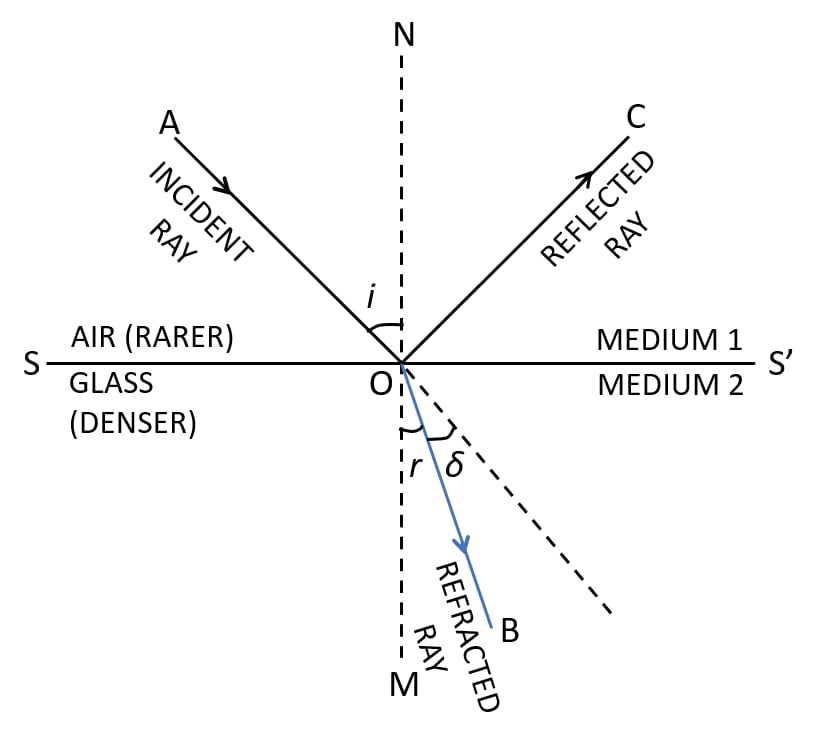(ii) The below ray diagram shows the refraction of light from glass to air: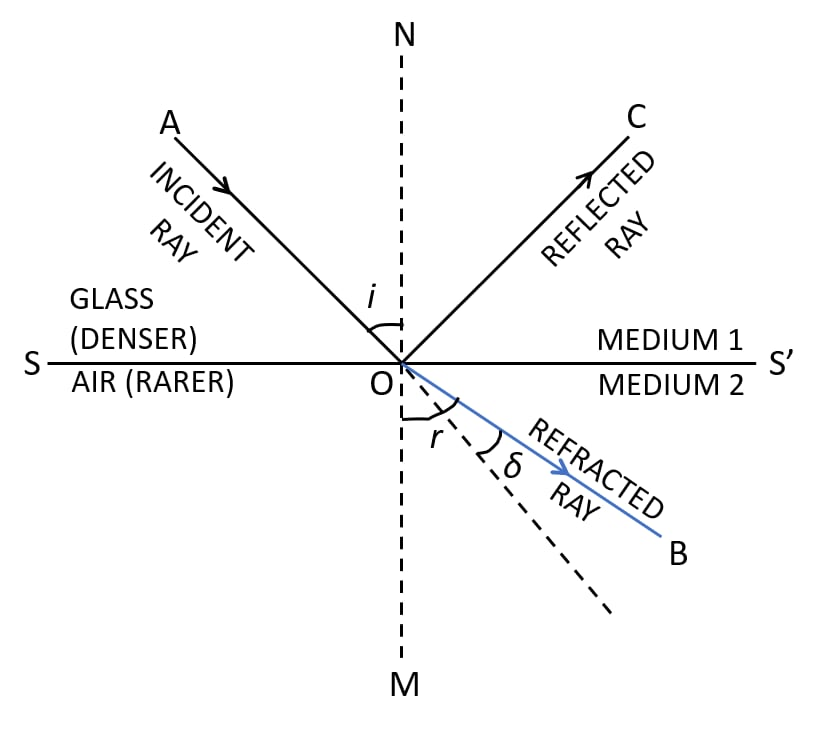#### Question 3

A ray of light is incident normally on a plane glass slab. What will be (i) the angle of refraction and (ii) the angle of deviation for the ray?

(i) The ray of light incident normally on a plane glass slab passes undeviated, ( i.e. such a ray suffers no bending at the surface). Thus, if angle of incidence ∠i = 0°, then the angle of refraction ∠r = 0°.

(ii) In the case when ray of light is incident normally on a plane glass slab, it passes undeviated. Hence, the angle of deviation is 0°.

#### Question 4

An obliquely incident light ray bends at the surface due to change in speed, when passing from one medium to other. The ray does not bend when it is incident normally. Will the ray have different speed in the other medium?

We observe that, when a ray of light passing from one medium to other is obliquely incident it bends at the surface due to change in speed in going from one medium to another.

However, for normal incidence from one medium to another, angle of incidence ∠i = 0° then the angle of refraction ∠r = 0°. Hence the speed of light changes but the direction of light does not change.

#### Question 5

What is the cause of refraction of light when it passes from one medium to another?

When a ray of light passes from one medium to another medium, its direction (or path) changes because of the change in speed of light in going from one medium to another.

In passing from one medium to other, if light slows down, it bends towards the normal and if light speeds up, it bends away from the normal.

If the speed of light remains same in passing from one medium to another, the ray of light does not bend then it passes undeviated.

Hence, we can say that the cause of refraction of light is that its speed is different in different mediums.

#### Question 6

A light ray suffers reflection and refraction at the boundary in passing from air to water. Draw a neat labelled diagram to show it.

Air is a rarer medium while water is denser medium than air. Therefore, when a ray of light travels from air to water (i.e rarer to denser) it bends towards the normal. Below diagram shows reflection and refraction suffered by a light ray at the boundary in passing from air to water: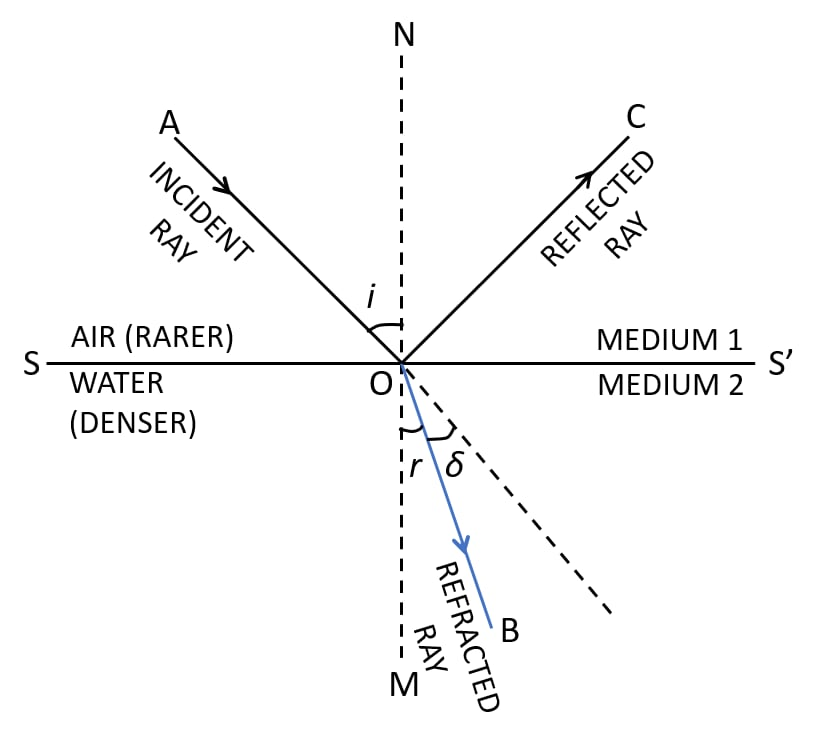#### Question 7

A ray of light passes from medium 1 to medium 2. Which of the following quantities of the refracted ray will differ from that of the incident ray: speed, intensity, frequency and wavelength?

Speed, intensity and wavelength are the quantities which will differ for a refracted ray in comparison to those of a incident ray.

The frequency of light depends on the source of light, so it does not change on refraction.

When a ray of light gets refracted from a rarer to a denser medium, the speed of light decreases; while if it is refracted from a denser to a rarer medium, the speed of light increases.

When light passes from a rarer to a denser medium, the wavelength decreases and when it passes from denser to rarer medium, its wavelength increases.

#### Question 8

State the Snell’s laws of refraction of light.

The Snell’s laws of refraction of light are:

(i) The incident ray, the refracted ray and the normal at the point of incidence, all lie in the same plane.

(ii) The ratio of the sine of the angle of incidence i to the sine of the angle of refraction r is constant for the pair of given media.

$\dfrac{\text{sin i}}{\text{sin r}} = \text{constant } _1\mu_2 \\[0.5em]$

The constant 1μ2 is called the refractive index of the second medium with respect to the first medium.

#### Question 9

Define the term refractive index of a medium. Can it be less than 1?

The refractive index of the second medium with respect to the first medium is defined as the ratio of the sine of the angle of incidence in the first medium to the sine of the angle of refraction in the second medium.

$μ = \dfrac{\text{Speed of light in vacuum or air(c)}}{\text{Speed of light in that medium (V)}}$

The refractive index of a transparent medium is always greater than 1 (it cannot be less than 1) because the speed of light in any medium is always less than that in vacuum ( i.e. V < c ) and μ = 1 for air or vacuum.

#### Question 10

(a) Compare the speeds of light of wavelength 4000Å (i.e. violet light) and 8000Å (i.e. red light) in vacuum.

(b) How is the refractive index of a medium related to the speed of light in it and in vacuum or air?

(a) As we know that light is an electromagnetic wave and it travels with same speed for all wavelength, which is equal to 3 x 108.

Hence, the ratio of speeds of light of wavelength 4000Å (i.e. violet light) and 8000Å (i.e. red light) in vacuum = 1:1.

(b) The refractive index of a medium is defined as the ratio of the speed of light in vacuum (or air) to the speed of light in that medium.

$μ =\dfrac{\text{Speed of light in vacuum or air (c) }}{\text {Speed of light in that medium (V)}} \\[0.5em]$

Denser medium has a higher refractive index. Hence, the speed of light in such medium is lower in comparison to the speed of light in a medium which has a lower refractive index.

#### Question 11

A light ray passes from water to (i) air, and (ii) glass. In each case, state how does the speed of light change?

As we know,

Refractive index of air, μa = 1.0003

Refractive index of water, μw = 1.33

Refractive index of ordinary glass, μg = 1.5

With the help of the above values, we can see that μg > μw > μa

When a ray of light gets refracted from a rarer to a denser medium, the speed of light decreases, while if it is refracted from a denser to a rarer medium, the speed of light increases.

So on the basis of above information, we can say that —

(i) When a ray of light passes from water to air, its speed increases as it is moving from a denser to a rarer medium.

(ii) When a ray of light passes from water to glass, its speed decreases as it is moving from a rarer to a denser medium.

#### Question 12

A light ray in passing from water to a medium (a) speeds up (b) slows down. In each case, (i) give one example of the medium, (ii) state whether the refractive index of medium is equal to, less than or greater than the refractive index of water.

As we know that, when a ray of light gets refracted from a rarer to a denser medium, the speed of light decreases, while if it is refracted from a denser to a rarer medium, the speed of light increases.

(a) (i) When a ray of light passes from water to air, its speed increases as it is moving from a denser to a rarer medium.

(b) (i) When a ray of light passes from water to glass, its speed decreases as it is moving from a rarer to a denser medium.

(a) (ii) The refractive index of the medium (air) is less than that of water as the speed of refracted ray increases.

(b) (ii) The refractive index of the medium (glass) is more than that of water as the speed of refracted ray decreases.

#### Question 13

What do you understand by the statement 'the refractive index of glass is 1.5 for white light'?

This statement indicates that white light travels in air 1.5 times faster than in glass or speed of light in glass is $\dfrac{1}{1.5}$ times the speed of light in air.

#### Question 14

A monochromatic ray of light passes from air to glass. The wavelength of light in air is λ, the speed of light in air is c and in glass is V. If the refractive index of glass is 1.5, write down,

(a) the relationship between c and V,

(b) the wavelength of light in the glass.

(a) The relation between the speed of light in air c and in glass V is given by —

$μ = \dfrac{\text{Speed of light in vacuum or air (c) }}{\text {Speed of light in that medium (V)}} \\[0.5em] 1.5 = \dfrac{c}{V} \\[0.5em] \Rightarrow c = 1.5 V \\[0.5em]$

(b) Let wavelength of light in glass be λ1 so we get,

$μ = \dfrac{λ}{λ_1} \\[0.5em] 1.5 = \dfrac{λ}{λ_1} \\[0.5em] λ_1 = \dfrac{λ}{1.5} \\[0.5em]$

#### Question 15

A boy uses blue colour of light to find the refractive index of glass. He then repeats the experiment using red colour of light. Will the refractive index be the same or different in the two cases? Give a reason to support your answer.

The speed of blue light in glass is less that of red light.

i.e. cb < cr

We know that,

$μ = \dfrac{\text{Speed of light in vacuum or air (c) }}{\text {Speed of light in glass (V)}} \\[0.5em]$

Hence, the refractive index of blue light is greater than that of red light.

i.e. μb > μr.

Hence, the refractive index of red and blue light in glass will be different.

#### Question 16a

For which colour of white light, is the refractive index of a transparent medium

(i) the least

(ii) the most?

(i) The speed of red light is maximum. Therefore, the refractive index of a transparent medium is least for red colour.

(ii) The speed of violet light is least. Therefore, the refractive index of a transparent medium is most for violet colour.

#### Question 16b

Which colour of light travels fastest in any medium except air?

The wavelength of visible light increases from violet to red colour. It is highest for red colour. Hence, red colour travels fastest in any medium except air.

#### Question 17

Name two factors on which the refractive index of a medium depends? State how does it depends on the factors stated by you.

The factors on which the refractive index of a medium depends are as follows —

(i) Nature of the medium (on the basis of speed of light) — Less the speed of light in the medium as compared to that in air, more is the refractive index of the medium.

We know that,

$μ = \dfrac{\text{Speed of light in vacuum or air (c) }}{\text {Speed of light in glass (V)}} \\[0.5em]$

Vglass = 2 x 10 8 ms-1, μglass = 1.5

Vwater = 2.25 x 10 8 ms-1, μwater = 1.33

(ii) Physical condition such as temperature — With an increase in temperature, the speed of light in medium increases, so the refractive index of the medium decreases.

#### Question 18

How does the refractive index of a medium depend on the wavelength of light used?

In a given medium, the speed of red light is maximum. Therefore, the refractive index of that medium is maximum for violet light and least for red light. (i.e. μviolet > μred).

The wavelength of visible light increases from violet to red end, so the refractive index of a medium decreases with increase in wavelength of light used.

Hence, the refractive index of a medium for violet light which has the least wavelength is greater than that for red light which has the greatest wavelength.

#### Question 19

How does the refractive index of a medium depend on its temperature?

With increase in temperature, the speed of light in a medium increases, so the refractive index of the medium decreases.

#### Question 20

Light of a single colour is passed through a liquid having a piece of glass suspended in it. On changing the temperature of the liquid, at a particular temperature, the glass piece is not seen.

(i) When is the glass piece not seen?

(ii) Why is the light of a single colour used?

(i) The glass piece is not seen when the refractive index of the liquid becomes equal to the refractive index of glass.

(ii) The light of a single colour is used as the refractive index of a medium (glass or liquid) is different for the light of different colours.

#### Question 21

In the figure below, a ray of light A incident from air suffers partial reflection and refraction at the boundary of water.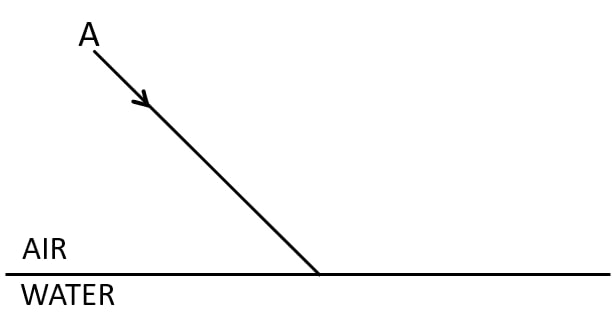(a) Complete the diagram showing (i) the reflected ray B and (ii) the refracted ray C.

(b) How are the angles of incidence i and refraction r related?

(a) Below is the completed diagram with the refracted ray B and the reflected ray C labelled:(b) The relation between angle of incidence i and the angle of refraction r is given by Snell’s law as,

The ratio of the sine of the angle of incidence i to the sine of the angle of refraction r is constant for the pair of given media.

$\dfrac{\text{Sin i}}{\text{sin r}} = \text {constant } _1\mu_2 \\[0.5em]$

The constant 1μ2 is called the refractive index of the second medium with respect to the first medium

$\dfrac{\text{sin i}}{\text{sin r}} = \mu w$

#### Question 22

The diagram alongside shows the refraction of a ray of light from air to a liquid.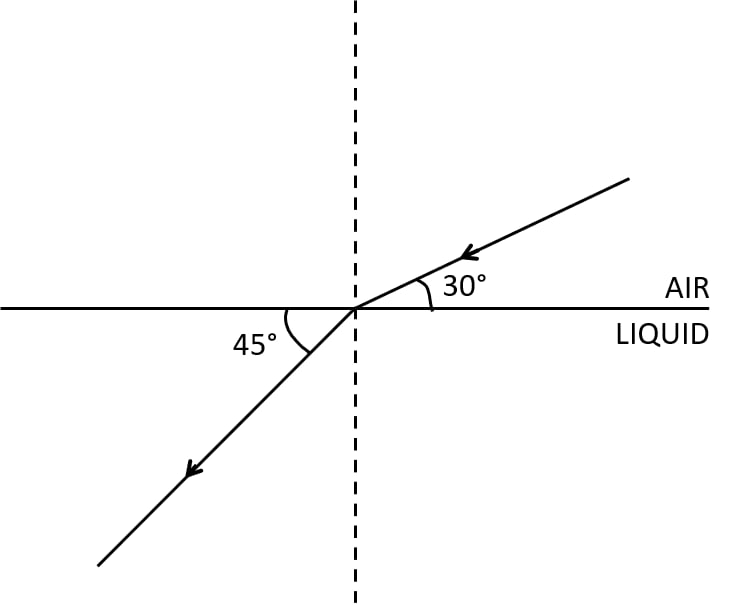(a) Write the values of (i) angle of incidence, and (ii) angle of refraction.

(b) Use Snell's law to find the refractive index of liquid with respect to air.

(a) (i) The angle of incidence is the angle which the incident ray makes with the normal. Hence, ∠i = 90° – 30° = 60°.

(ii) Angle of refraction is the angle which the refracted ray makes with the normal Hence, ∠r = 90° – 45° = 45°.

(b) We know that according to Snell’s law,

$_{\text{air}}\text{μ}_{\text{liquid}} = \dfrac{\text{sin i}}{\text{sin r}} \\[0.5em] = \dfrac{\text{sin 60} \degree}{\text{sin 45} \degree} \\[0.5em] = \dfrac{\dfrac{\sqrt{3}}{2}}{\dfrac{1}{\sqrt{2}}} \\[0.5em] = \dfrac{\sqrt{3}}{\sqrt{2}} \\[0.5em] = 1.22$

Hence, the refractive index of liquid with respect to air is 1.22

#### Question 23

The refractive index of water with respect to air is aμw and of glass with respect to air is aμg. Express the refractive index of glass with respect to water.

The refractive index of water with respect to air is aμw.

$_{\text{a}}\text{μ}_{\text{w}} = \dfrac{\text{Speed of light in air}}{\text {Speed of light in water}} \\[0.5em]$

The refractive index of glass with respect to air is aμg.

$_{\text{a}}\text{μ}_{\text{g}} = \dfrac{\text{Speed of light in air}}{\text {Speed of light in glass}} \\[0.5em]$

So, the refractive index of glass with respect to water is given by

$_{\text{w}}\text{μ}_{\text{g}} = \dfrac{_a\mu_g}{_a\mu_w} \\[1em]$

#### Question 24

What is lateral displacement? Draw a ray diagram showing the lateral displacement of a ray of light when it passes through a parallel-sided glass slab.

The perpendicular distance between the incident ray and the emergent ray is defined as lateral displacement. This shift depends upon the angle of incidence, the angle of refraction and the thickness of the medium.

Below diagram shows the lateral displacement of a ray of light when it passes through a parallel-sided glass slab: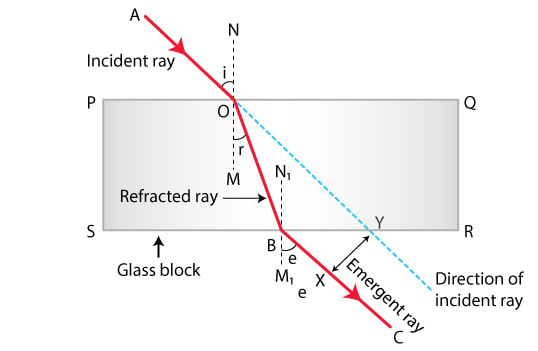#### Question 25

A ray of light strikes the surface at a rectangular glass slab such that the angle of incidence in air is (i) 0°, (ii) 45°. In each case, draw a diagram to show the path taken by the ray as it passes through the glass slab and emerges from it.

(i) Below is the labelled diagram for ray of light at angle of incidence 0°: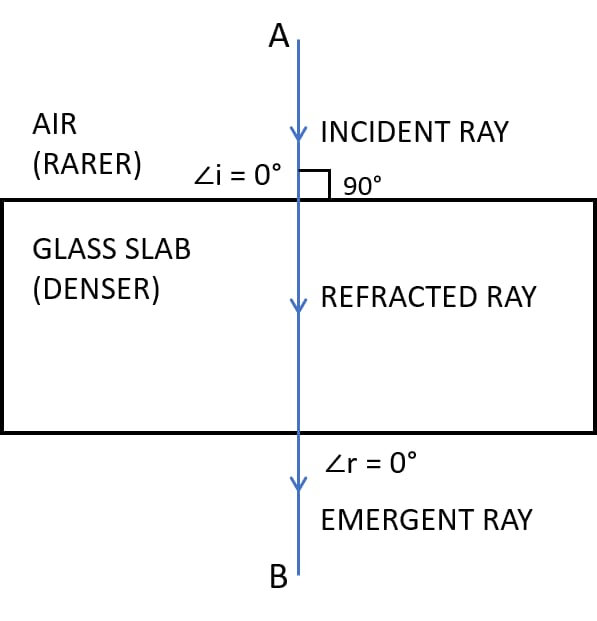(ii) Below is the labelled diagram for ray of light at angle of incidence 45°: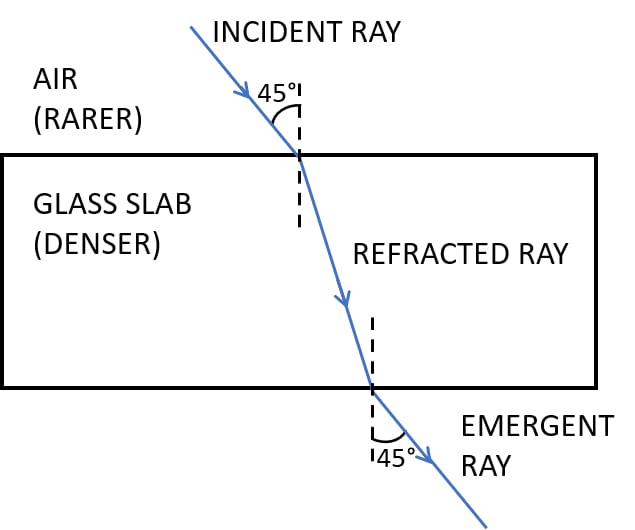#### Question 26

In the adjacent diagram, AO is a ray of light incident on a rectangular glass slab.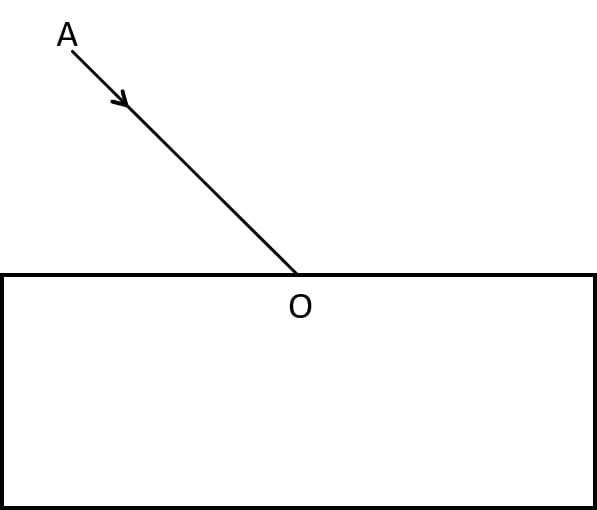(a) Complete the path of the ray until it emerges out of the slab.

(b) In the diagram, mark the angle of incidence (i) and the angle of refraction (r) at the first interface. How is the refractive index of glass related to angles i and r?

(c) Mark angle of emergence by the letter e. How are the angles i and e related?

(d) Which two rays are parallel to each other? Name them.

(e) Indicate in the diagram the lateral displacement between the emergent ray and the incident ray. State one factor that affects the lateral displacement.

(a) The below diagram shows the path of the ray until it emerges out of the slab:(b) In diagram, the angle of incidence (i) and the angle of refraction (r) are marked.

Refractive index of glass μ is related to the angle of incidence (i) and angle of reflection (r) by Snell's second law and is represented as —

The ratio of the sine of the angle of incidence i to the sine of the angle of refraction r is constant for the pair of given media

$\dfrac{\text{sin i}}{\text{sin r}} = \text{constant } _1\mu_2 \\[0.5em]$

The constant 1μ2 is called the refractive index of the second medium with respect to the first medium.

(c) The angle of emergence (e) is marked in diagram.

As the refraction occurs at two parallel surfaces PQ and RS, therefore angle MOB = angle N1BO and by the principle of reversibility of the path of a light ray angle of emergence (e) is equal to the angle of incidence (i).

(d) As the refraction occurs at two parallel surfaces PQ and RS, therefore angle MOB = angle N1BO and by the principle of reversibility of the path of a light ray angle of emergence (e) is equal to the angle of incidence (i).

Hence, the emergent ray BC is parallel to the incident ray AO.

(e) The lateral displacement between the incident ray and the emergent ray is represented by XY.

One of the factors that affects lateral displacement is the the thickness of the medium. More the thickness of the medium more is the lateral displacement.

#### Question 27

A ray of green light enters a liquid from air, as shown in the figure. The angle 1 is 45° and angle 2 is 30°.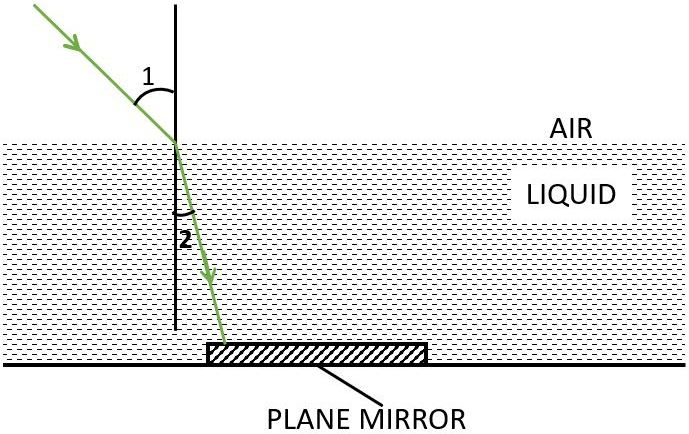(a) Find the refractive index of liquid.

(b) Show in the diagram the path of the ray after it strikes the mirror and re-enters in air. Mark in the diagram the angles wherever necessary.

(c) Redraw the diagram if plane mirror becomes normal to the refracted ray inside the liquid. State the principle used.

(a) Refractive index of the liquid is given by Snell's law and is shown as below,

airμliquid = $\dfrac{\text{sin i}}{\text{sin r}}$

airμliquid = $\dfrac{\text{sin 45}\degree}{\text{sin 30}\degree}$

$= \dfrac{\dfrac{1}{\sqrt{2}}}{\dfrac{1}{2}} \\[0.5em] = \dfrac{2}{\sqrt{2}} \\[0.5em] = \sqrt{2} \\[0.5em] = 1.414 \\[0.5em]$

(b) Below labelled diagram shows the path of the ray after it strikes the mirror and re-enters in air: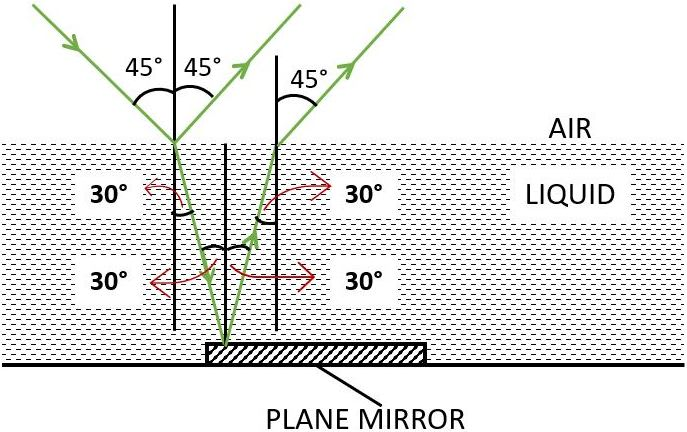(c) Below labelled diagram shows the path of the ray when it strikes the plane mirror normally inside the liquid: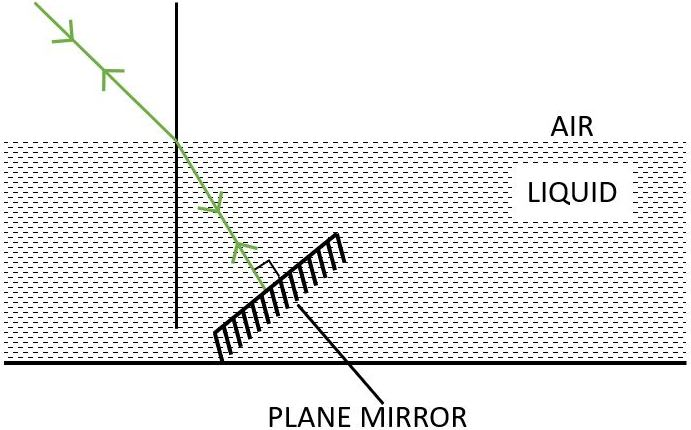The principle of reversibility is used.

#### Question 28

When an illuminated object is held in front of a thick plane glass mirror, several images are seen, out of which the second image is the brightest. Give reason.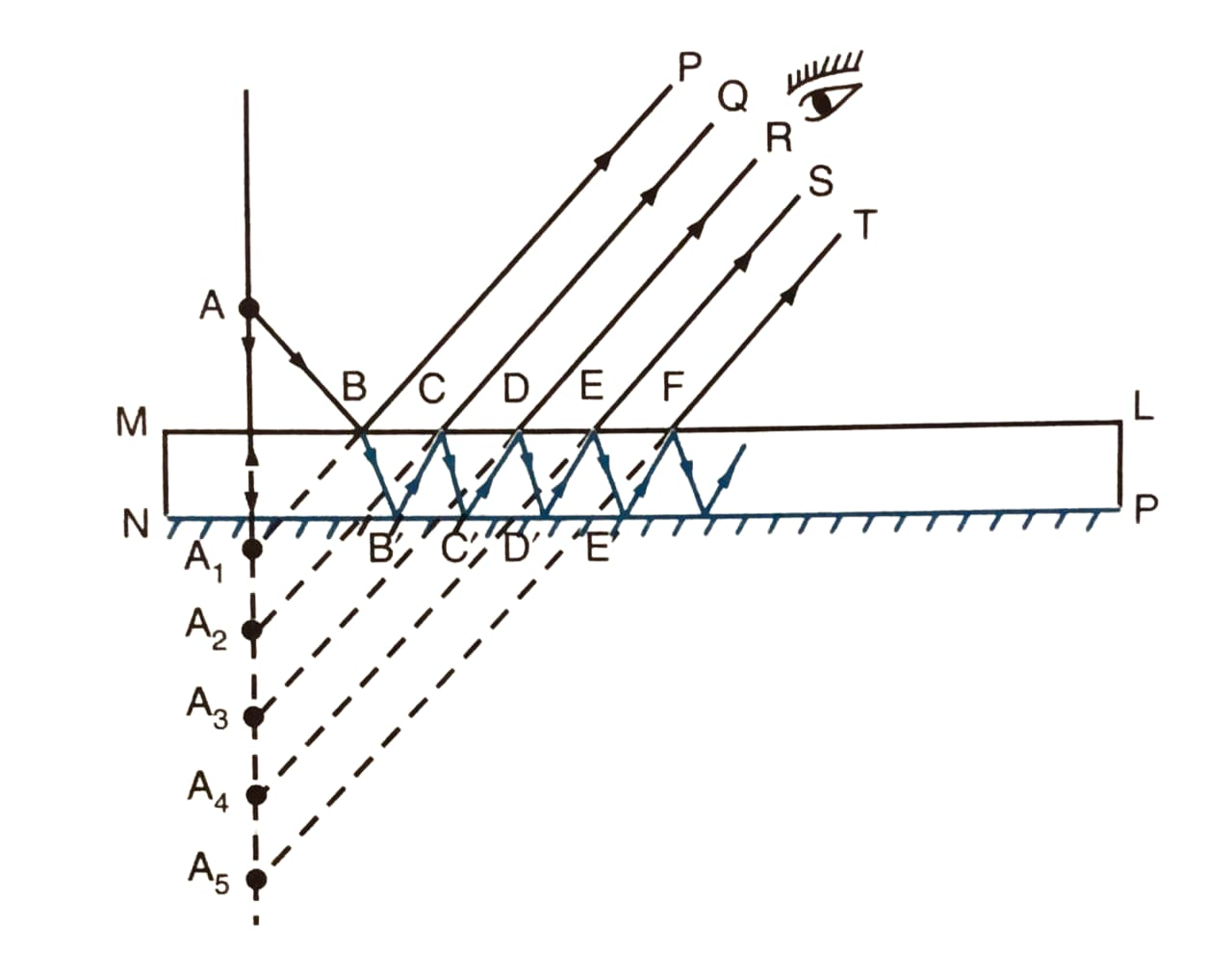As shown in the figure, if an illuminated object is held in front of a thick plane glass mirror, and is viewed obliquely, a number of images are seen and out of these the second image is the brightest.

The reason for this is that when a ray of the light falls on the surface of the mirror from the source, a small part of light (nearly 4%) is reflected forming a faint virtual image, while a large part of light (nearly 96%) is refracted inside the glass.

Now, we can see that this ray is strongly reflected back by the silvered surface inside the glass. This ray is then partially refracted in air and this refracted ray forms another virtual image.

This image is the brightest image because it is due to the light suffering a strong first reflection at the silvered surface.

#### Question 29

Fill in the blanks to complete the following sentences —

(a) When light travels from a rarer to a denser medium, its speed ………………….

(b) When light travels from a denser to a rarer medium, its speed ………………….

(c) The refractive index of glass with respect to air is 3/2. The refractive index of air with respect to glass will be ………………….

(a) When light travels from a rarer to a denser medium, its speed decreases.

(b) When light travels from a denser to a rarer medium, its speed increases.

(c) The refractive index of glass with respect to air is 3/2. The refractive index of air with respect to glass will be 2/3.

## Multiple Choice Type

#### Question 1

When a ray of light from air enters a denser medium, it:

1. bends away from the normal
2. bends towards the normal ✓
3. goes undeviated
4. is reflected back

#### Question 2

A light ray does not bend at the boundary in passing from one medium to the other medium if the angle of incidence is:

1. 0° ✓
2. 45°
3. 60°
4. 90°

#### Question 3

The highest refractive index is of:

1. Glass
2. Water
3. Diamond ✓
4. Ruby

## Numericals

#### Question 1

The speed of light in air is 3 x 108 m s-1. Calculate the speed of light in glass. The refractive index of glass is 1.5.

As we know,

$μ = \dfrac{\text{Speed of light in vacuum or air (c) }}{\text {Speed of light in glass (V)}} \\[0.5em]$

Given,

Refractive index of glass, μ = 1.5

Speed of light in air, C = 3 × 108 m / s

Substituting the values in the formula above we get,

$1.5 = \dfrac{3 \times 10^8 }{V} \\[0.5em] V = \dfrac{3 \times 10^8}{1.5} \\[0.5em] \Rightarrow V = 2 \times 10^8$

Hence, Speed of light in glass = 2 × 108 m s-1

#### Question 2

The speed of light in diamond is 125,000 km s-1. What is the refractive index? (Speed of light in air = 3 x 108 m s-1).

As we know,

$μ = \dfrac{\text{Speed of light in vacuum or air (c) }}{\text {Speed of light in diamond (V)}} \\[0.5em]$

Given,

Speed of light in diamond = 125,000 km s-1 = 125 x 106 m/s

Speed of light in air, c = 3 × 108 ms-1

Substituting the values in the formula above we get,

$μ = \dfrac{3 \times 10^8 }{125 \times 10^6} \\[0.5em] μ = \dfrac{3 \times 10^2}{125} \\[0.5em] μ = \dfrac{300}{125} \\[0.5em] \Rightarrow μ = 2.4 \\[0.5em]$

Hence, refractive index = 2.4

#### Question 3

The refractive index of water with respect to air is 4/3. What is the refractive index of air with respect to water?

As it is given that,

aμw = $\dfrac{4}{3}$

And we know that —

wμa = $\dfrac{1}{\dfrac{4}{3}}$

wμa = $\dfrac{3}{4}$

wμa = 0.75

Hence, the refractive index of air with respect to water is 0.75

#### Question 4

A ray of light of wavelength 5400 Å suffers refraction from air to glass. Taking aμg = 3/2, find the wavelength of light in glass.

As we know that,

$_{\text{a}}\text{μ}_{\text{g}} = \dfrac{\text {wavelength of light in air}}{\text {wavelength of light in glass}} \\[0.5em]$

Given,

Refractive index of glass with respect to air is given by aμg = $\dfrac{3}{2}$

Wavelength of light in air = 5400 Å

Substituting the values in the formula above we get,

$\dfrac{3}{2} = \dfrac{\text {wavelength of light in air}}{\text{wavelength of light in glass}} \\[0.5em] \dfrac{3}{2} = \dfrac{5400}{\text {wavelength of light in glass}} \\[0.5em] \text {wavelength of light in glass} = \dfrac{5400}{\dfrac{3}{2}} \\[0.5em] \text {wavelength of light in glass} = 5400 \times \dfrac{2}{3} \\[0.5em] \text {wavelength of light in glass} = 3600 \\[0.5em]$

Hence, wavelength of light in glass = 3600 Å

## Exercise 4(B)

#### Question 1

What is a prism? With the help of a diagram of the principal section of a prism, indicate its refracting surfaces, refracting angle and base.

A prism is defined as a transparent medium bounded by five plane surfaces with a triangular cross section. The below diagram shows the principal section of a prism with its refracting surfaces, refracting angle and base labelled: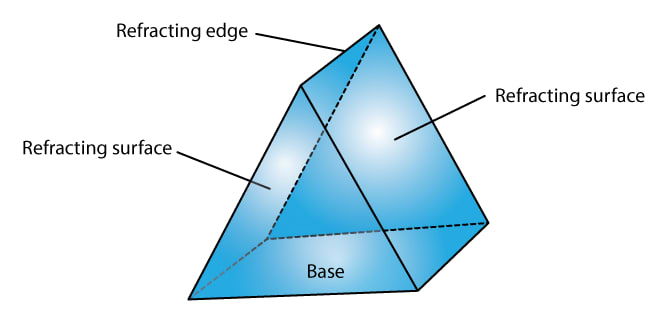#### Question 2

The diagrams (a) and (b) in figure below show the refraction of a ray of light of single colour through a prism and a parallel sided glass slab, respectively.

(a)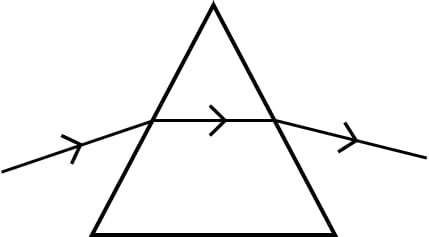(b)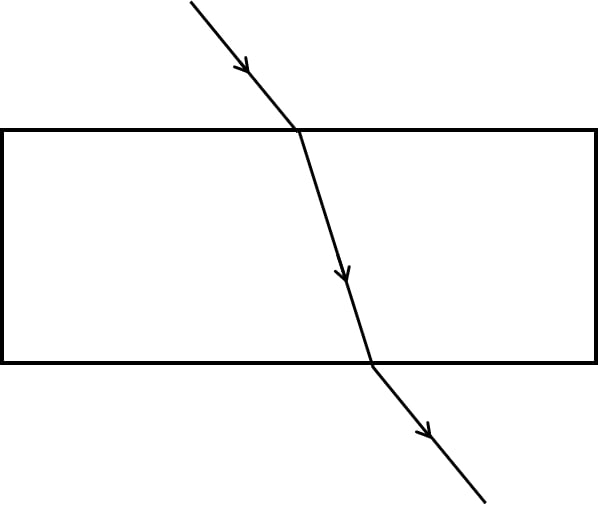(i) In each diagram, label the incident, refracted, emergent rays and the angle of deviation.

(ii) In what way the direction of the emergent ray in the two cases differ with respect to the incident ray? Explain your answer.

(i) The below diagram shows the refraction of a ray of light of single colour through a prism with the incident, refracted, emergent rays and the angle of deviation properly labelled: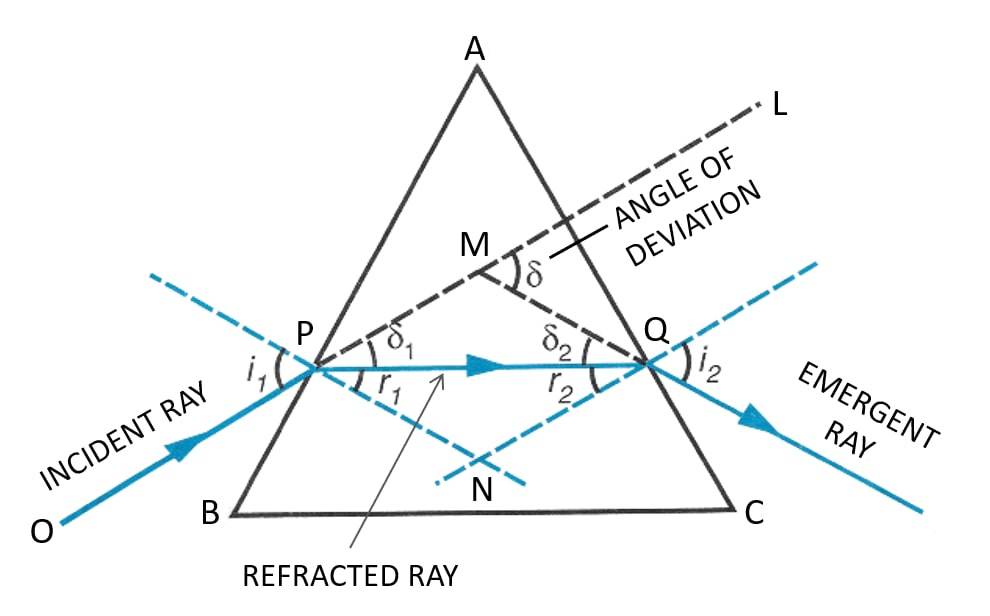The below diagram shows the refraction of a ray of light of single colour through a parallel sided glass slab with the incident, refracted, emergent rays and the angle of deviation properly labelled:(ii) In a prism, the refraction of light occurs at two inclined faces, so the emergent ray is not parallel to the incident ray but it is deviated towards the base of the prism. On the other hand, in a parallel sided glass slab, the refraction of light occurs at two parallel faces, so the emergent ray is parallel to the incident ray with a lateral displacement.

#### Question 3

Define the term angle of deviation.

Angle of deviation is the angle between the direction of incident ray and the emergent ray. It is represented by the greek alphabet δ (delta).

#### Question 4

Complete the following sentence:

Angle of deviation is the angle which the ...... ray makes with the direction of ...... ray.

Angle of deviation is the angle which the emergent ray makes with the direction of incident ray.

#### Question 5

What do you understand by the deviation produced by a prism? Why is it caused? State three factors on which the angle of deviation depends.

In a prism, the ray of light suffers refraction at two inclined faces. In each refraction, the ray bends towards the base of the prism. The below diagram shows the refraction of a ray of light of single colour through a prism:At the first face AB, the ray suffers a deviation equal to δ1. Then, at the second face AC, the ray PQ suffers a deviation δ2. Thus, the prism has produced a deviation which is the angle between the direction of incident ray and the emergent ray. This is called the angle of deviation produced by a prism.

The angle of deviation is caused as the ray passing through a prism suffers refraction at two inclined planes.

The three factors on which the angle of deviation depends are as follows:

1. The angle of incidence (i)
2. The material of prism (i.e on refractive index μ)
3. The angle of prism (A)

#### Question 6

(a) How does the angle of deviation produced by a prism change with increase in the angle of incidence. Draw a curve showing the variation in the angle of deviation with the angle of incidence at a prism surface.

(b) Using the curve in part (a) above, how would you infer that for a given prism, the angle of minimum deviation 𝛿min is unique for light of a given wavelength.

(a) It is experimentally observed that as the angle of incidence increases, the angle of deviation first decreases, reaches to a minimum value for a certain angle of incidence and then on further increasing the angle of incidence, the angle of deviation begins to increase. The below curve shows the variation in the angle of deviation with the angle of incidence at a prism surface: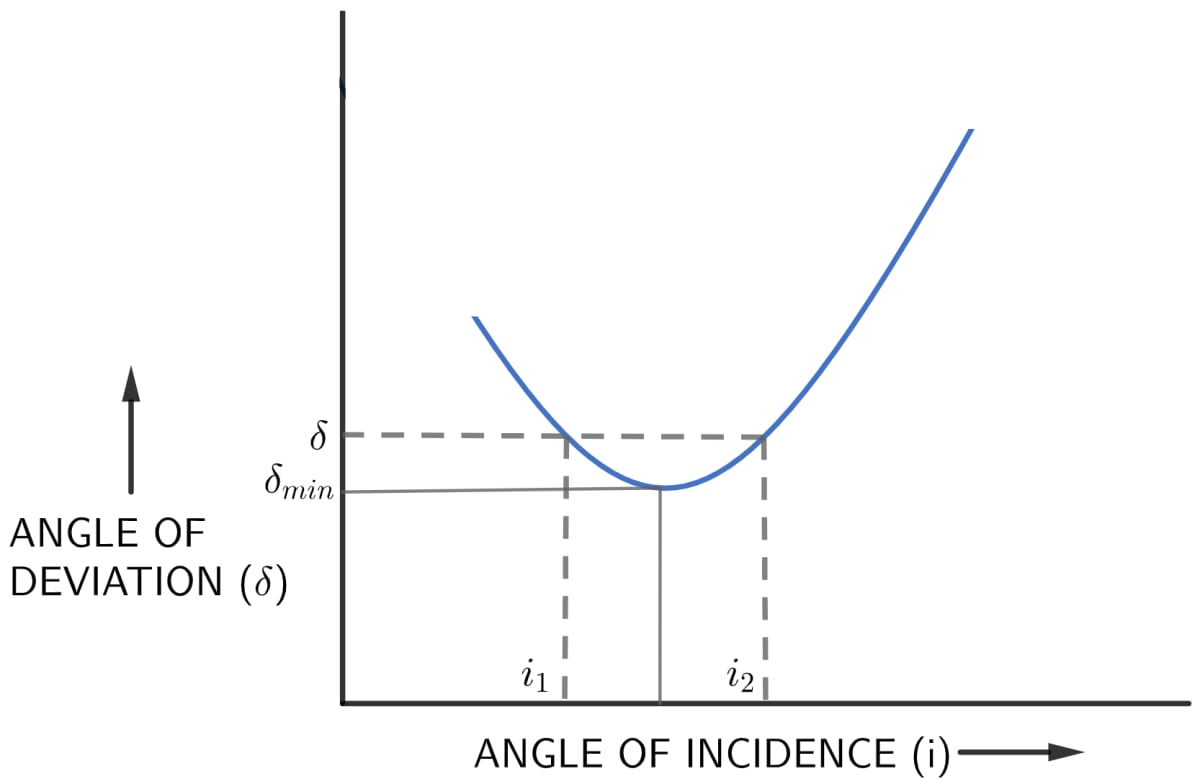(b) For a given prism and given colour of light, 𝛿min (angle of minimum deviation) is unique since only one horizontal line can be drawn parallel to i – axis at the lowest point of i – 𝛿 curve i.e only for one value of angle of incidence i, the refracted ray inside the prism is parallel to its base.

#### Question 7

State whether the following statement is 'true' or 'false'.

The deviation produced by a prism is independent of the angle of incidence and is same for all the colours of light.

The statement given above is False.

#### Question 8

How does the deviation produced by a prism depend on

(i) the refractive index of its material, and

(ii) the wavelength of incident light

(i) For a given angle of incidence, the prism with a higher refractive index produces greater deviation than the prism which has a lower refractive index.

For example — A flint glass prism produces more deviation than a crown glass prism for same refracting angle since μflint > μcrown

(ii) The refractive index of a given transparent medium is different for the light of different colours. It decreases with the increase in the wavelength of light.

Thus, the refractive index of the material of a prism for visible light is maximum for the violet colour and minimum for the red colour.

Consequently, a given prism deviates violet light the most and red light the least.

(i.e. δ violet > δ red since μviolet > μred )

#### Question 9

How does the angle of minimum deviation produced by a prism change with increase in (i) the wavelength of incident light and (ii) the refracting angle of the prism?

(i) The angle of minimum deviation produced by a prism decreases with increase in the wavelength of incident light.

(ii) The angle of minimum deviation produced by a prism increases with increase in the refracting angle of the prism.

#### Question 10

Write a relation for the angle of deviation (𝛿) for a ray of light passing through an equilateral prism in terms of angle of incidence (i1), angle of emergence (i2) and angle of prism (A).

The relation between the angle of incident (i1), angle of emergence (i2), angle of prism (A) and angle of deviation (𝛿) for a ray of light passing through an equilateral prism is given by —

𝛿 = i1 + i2 - A

#### Question 11

A ray of light incident at an angle of incidence i1 passes through an equilateral glass prism such that the refracted ray inside the prism is parallel to its base and emerges at an angle of emergence i2.

(i) How is the angle of emergence 'i2' related to the angle of incidence 'i1'.

(ii) What can you say about the angle of deviation in such a situation?

(i) When a ray of light incident at an angle of incidence i1 passes through an equilateral glass prism such that the refracted ray inside the prism is parallel to its base and emerges at an angle of emergence i2 then angle of incidence i1 is equal to the angle of emergence i2.

Hence,

i1 = i2

(ii) When a ray of light incident at an angle of incidence i1 passes through an equilateral glass prism such that the refracted ray inside the prism is parallel to its base and emerges at an angle of emergence i2 then angle of deviation is minimum.

#### Question 12

Draw a ray diagram to show the refraction of a monochromatic ray through a prism when it suffers minimum deviation. How is the angle of emergence related to the angle of incidence in this position.

Below diagram shows the refraction of a monochromatic light ray through a prism when it suffers minimum deviation: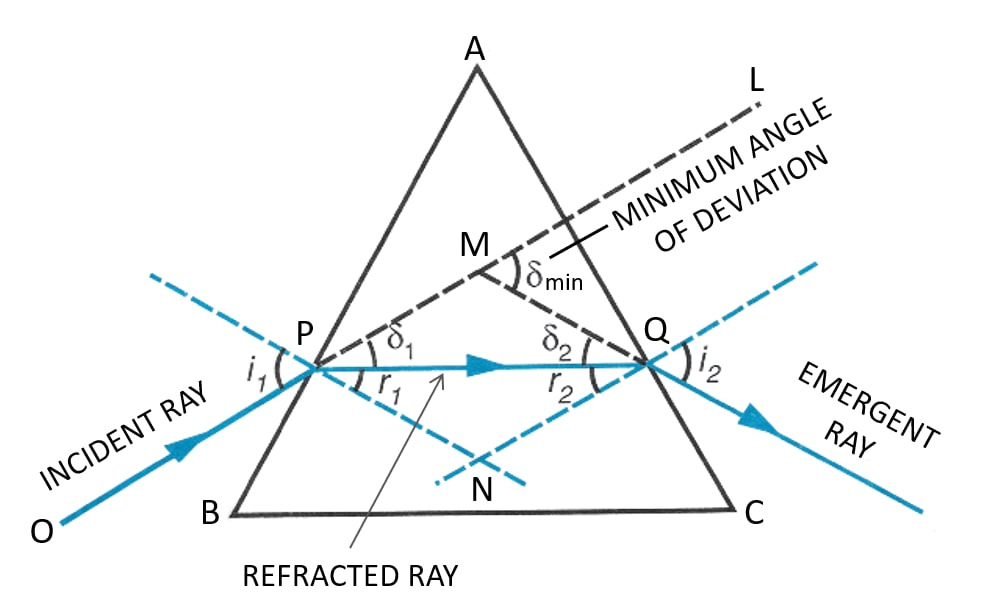In an equilateral prism, when the prism is in minimum deviation, the angle of incidence i1 is equal to the angle of emergence i2.

Hence,

Angle of emergence (i2) = Angle of incidence (i1).

#### Question 13

A light ray of yellow colour is incident on an equilateral glass prism at an angle of incidence equal to 48° and suffers minimum deviation by an angle of 36°.

(i) What will be the angle of emergence?

(ii) If the angle of incidence is changed to (a) 30°, (b) 60°, state in each case whether the angle of deviation will be equal to less than or more than 36°?

(i) As we know that in an equilateral glass prism the ray suffers minimum deviation.

So, angle of incidence i1 is equal to angle of emergence i2

Given, angle of incidence i1 = 48°

Applying the rule given above, we get,

i1 = i2
⇒ i2 = 48°

Hence, angle of emergence i2 = 48°

(ii) (a) As the minimum angle of deviation is 36° for yellow light, at an incident angle of 48° so for any angle of incidence other than 48° the angle of deviation will be more than 36°.

Hence, when the angle of incidence is changed to 30°, the angle of deviation will be more than 36°.

(b) As the minimum angle of deviation is 36° for yellow light, at an incident angle of 48° so for any angle of incidence other than 48° the angle of deviation will be more than 36°.

Hence, when the angle of incidence is changed to 60°, the angle of deviation will be more than 36°.

#### Question 14

Name the colour of white light which is deviated

(i) the most,

(ii) the least, on passing through a prism.

(i) On passing through a prism, violet colour will deviate the most.

(ii) On passing through a prism, red colour will deviate the least

#### Question 15

Which of the two prisms, A made of crown glass and B made of flint glass, deviates a ray of light more?

For a given angle of incidence, the prism with a higher refractive index produces greater deviation than the prism which has a lower refractive index.

As refractive index of flint glass prism (B) is more than a crown glass prism (A) (i.e. μflint > μcrown)

Hence, a flint glass prism (B) produces more deviation than a crown glass prism (A).

#### Question 16

How does the angle of deviation depend on refracting angle of the prism?

The angle of deviation (𝛿) increases with increase in the angle of prism (A).

#### Question 17

An object is viewed through a glass prism with its vertex pointing upwards. Draw a ray diagram to show the formation of its image as seen by the observer.

Below ray diagram shows the formation of the image of an object when seen by an observer through a glass prism with its vertex pointing upwards: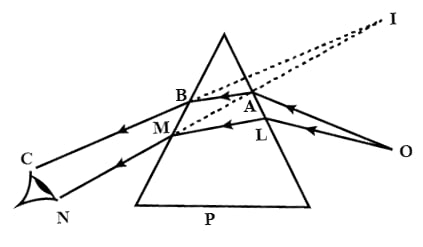#### Question 18

A ray of light is normally incident on one face of an equilateral glass prism.

Answer the following —

(a) What is the angle of incidence on the first face of the prism?

(b) What is the angle of refraction from the first face of the prism?

(c) What will be the angle of incidence at the second face of the prism?

(d) Will the light ray suffer minimum deviation by the prism?

(a) The angle of incidence on the first face of the prism when a ray of light is normally incident is 0°.

(b) The angle of refraction from the first face of the prism r1 = 0° as the angle of refraction of a normally incident ray is always 0°.

(c) As the prism is equilateral therefore A = 60°.

Given, r1 = 0°.

Hence, taking into consideration the two conditions we get,

the angle of incidence at the second face of the prism, equal to 60°.

(d) No, the light ray will not suffer minimum deviation as the angle of incidence is 0°.

#### Question 19

The diagram below shows two identical prisms A and B placed with their faces parallel to each other. A ray of light of single colour PQ is incident at the face of the prism A. Complete the diagram to show the path of the ray till it emerges out of the prism B.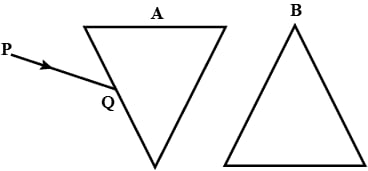[Hint: The emergent ray out of the prism B will be parallel to the incident ray PQ]

The below diagram shows the complete path of the ray as it enters prism A and emerges out of prism B: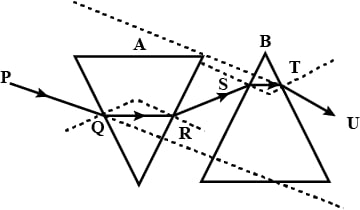## Multiple Choice Type

#### Question 1

In refraction of light through a prism, the light ray:

1. suffers refraction only at one face of the prism
2. emerges out from the prism in a direction parallel to the incident ray
3. bends at both the surfaces of the prism towards its base ✓
4. bends at both the surfaces of prism opposite to its base.

#### Question 2

A ray of light suffers refraction through an equilateral prism. The deviation produced by the prism does not depend on the:

1. angle of incidence
2. colour of light
3. material of prism
4. size of prism ✓

## Numericals

#### Question 1

A ray of light incident at an angle of incidence 48° on a prism of refracting angle 60° suffers minimum deviation. Calculate the angle of minimum deviation. [ Hint — δmin = 2i - A ]

As we know,

$δ_ {min} = 2i - A$

Given,

i = 48°

A = 60°

So, substituting the values in the formula above we get,

$δ_ {min} = (2 \times 48°) - 60° \\[0.5em] δ_ {min} = 96° - 60° \\[0.5em] \Rightarrow δ_ {min} = 36° \\[0.5em]$

Hence, the angle of minimum deviation is equal to 36°

#### Question 2

What should be the angle of incidence for a ray of light which suffers minimum deviation of 36° through an equilateral prism?[ Hint — A = 60° and i = ( A + δmin ) / 2 ]

As we know,

$δ_{min} = 2i - A$

Given,

$δ_{min} = 36°$

A = 60°

So, substituting the values in the formula above we get,

$36° = (2 \times i) - 60° \\[0.5em] \Rightarrow i = \dfrac{(36° + 60°)}{2} \\[0.5em] \Rightarrow i = \dfrac{96}{2} \\[0.5em] \Rightarrow i = 48° \\[0.5em]$

Hence, the angle of incidence is equal to 48°.

## Exercise 4(C)

#### Question 1

How is the refractive index of a medium related to the real and apparent depths of an object in that medium?

The relation of refractive index μ with real and apparent depth is given as below,

$_a\mu_m = \dfrac{\text{Real Depth}}{\text{Apparent Depth}}$

#### Question 2

Prove that,

$\text {Refractive index} = \dfrac {\text {Real depth}}{\text {Apparent depth}}$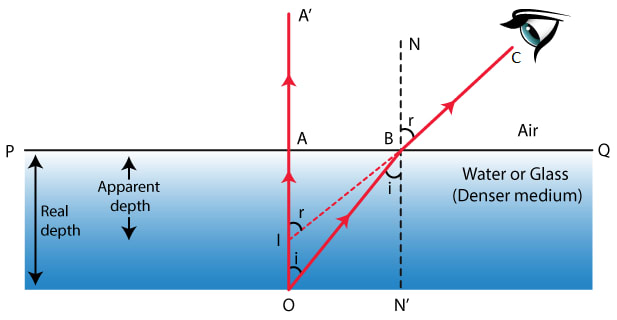Consider a point object O kept at the bottom of a transparent medium ( such as water or glass ) separated from air by the surface PQ.

A ray of light OA, starting from the object O, is incident on the surface PQ normally, so it passes undeviated along the path AA'. Another ray OB, starting along the object O, strikes the boundary surface PQ at B and suffers refraction.

Since, the ray travels from denser to rarer medium so it bends away from normal N'BN drawn at the point of incidence B on the surface PQ and travels along BC in air.

When viewed by the eye , the ray BC appears to be coming from point I which is the virtual image of O, obtained on producing A'A and CB backwards.

Thus, any point seen from air will appear to be at I, which is lesser depth = AI than its actual depth AO.

Angle of incidence = ∠OBN'

Angle of refraction = ∠CBN.

Since, AO and BN’ are parallel and OB is transversal line, so

∠AOB = ∠OBN' = i

Similarly, IA’ and BN are parallel and IC is the transversal line, so

∠BIA’ = ∠CBN = r

In right-angle triangle BAO,

$\text {sin i} = \dfrac{BA}{OB}$

and,

In right-angle triangle IAB,

$\text {sin r} = \dfrac{BA}{IB}$

For refraction from medium to air, by Snell’s law

$_mμ _a = \dfrac{\text {sin i}}{\text {sin r}} = \dfrac {\dfrac {BA}{OB}}{\dfrac {BA}{IB}} \\[0.5em] \Rightarrow _mμ _a = \dfrac{IB}{OB} \\[0.5em]$ Hence, refractive index of medium with respect to air is,

$_aμ _m = \dfrac{1}{_mμ_a} = \dfrac{OB}{IB} \\[0.5em]$

The object is viewed from a point vertically above the object O, since point B is very close to the point A. ∴ IB = IA and OB = OA

Hence,

$_aμ _m = \dfrac{1}{_mμ_a} = \dfrac{OA}{IA} \\[0.5em] \Rightarrow \dfrac{\text {Real depth}}{\text {Apparent depth}} \\[0.5em]$

#### Question 3

A tank of water is viewed normally from above.

(a) State how does the depth of tank appears to change.

(b) Draw a labelled ray diagram to explain your answer.

(a) When a tank is viewed normally from above from a rarer medium, the apparent depth of the tank is always less than its real depth.

As aμw = 4 / 3, therefore, the depth of the pond appears three fourth of its real depth on seeing it from air in a nearly vertical direction.

(b) Below is the labelled ray diagram of a tank of water viewed normally from above:#### Question 4

Water in a pond appears to be only three-quarters of its actual depth.

(a) What property of light is responsible for this observation? Illustrate your answer with the help of a ray diagram.

(b) How is the refractive index of water calculated from its real and apparent depths?

(a) Water in a pond appears to be only three-quarters of its actual depth due to the refraction of light while travelling from one medium to another.

The light rays suffer refraction while travelling from denser medium (water or glass) to rarer medium (air), so it bends away from the normal. Below is the ray diagram for this: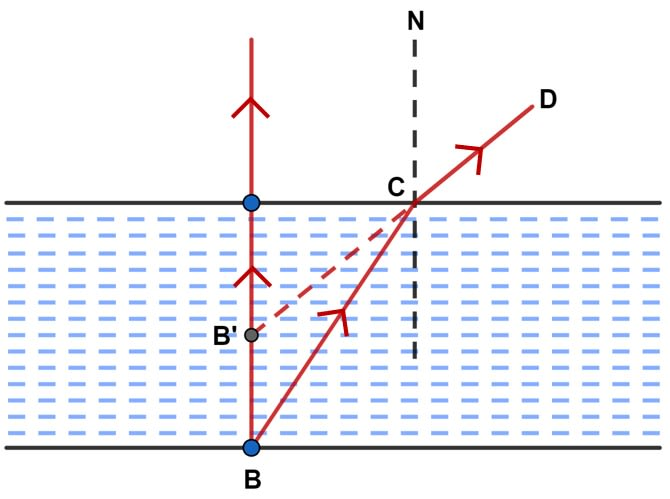(b) Let us say an object (B) is at the bottom of a pond. Consider a ray of light BC from the object that moves from water to air.

The ray now moves away from the normal N along the path CD, after refraction from the water surface.

When we produce CD it appears from the point B’.

The virtual image of the object B appears at B’.

Hence, we get,

$\text {Refractive index of water} = \dfrac {\text {Real depth}}{\text {Apparent depth}} \\[0.5em]$

#### Question 5

Draw a ray diagram to show the appearance of a stick partially immersed in water. Explain your answer.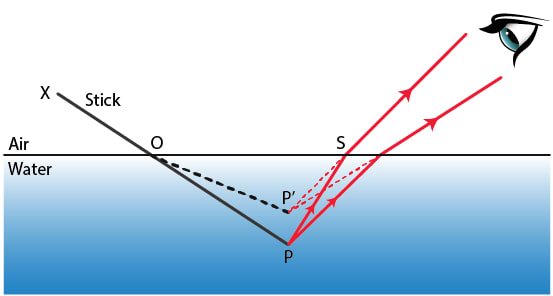The above figure shows a stick placed obliquely in water. The portion OP of the stick under water when seen from air appears to be shortened and raised up as OP'. This is due to refraction of light from water to air.

The rays of light coming from tip P of the stick when pass from water to air, bend away from the normal and appear to be coming from point P' which is the virtual image of the point P.

Thus, the part PO of the stick appears to be P'O.

Hence, the immersed part of the stick appears to be raised and therefore, bent at the point O at the surface of water and the stick XOP appears as XOP'.

#### Question 6

A fish is looking at a 1.0 m high plant at the edge of a pond. Will the plant appear to the fish shorter or taller than its actual height? Draw a ray diagram to support your answer.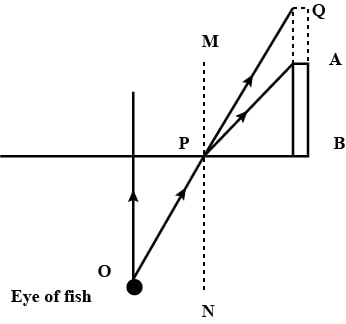When a fish is looking at a 1.0 m high plant at the edge of the pond, it appears to be taller than its actual height due to the refraction of the light rays travelling from one medium to another.

When the fish is looking at the plant, as air is a rarer medium in comparison to water, the ray OP will bend away from the normal while emerging out from the water to air.

But, when we extend the ray it appears to be coming from a higher point.

Hence, the plant will look taller than its actual height.

#### Question 7

A student puts his pencil into an empty trough and observes the pencil from the position as indicated in the figure.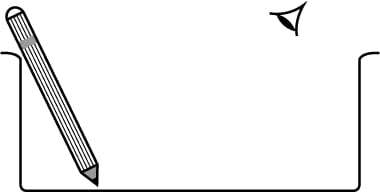(i) What change will be observed in the appearance of the pencil when water is poured into the trough?

(ii) Name the phenomenon which accounts for the above-started observation.

(iii) Complete the diagram showing how the student’s eye sees the pencil through water.

(a) When a student puts his pencil into an empty trough, and later pours water in the same trough, the part of the pencil which is immersed in water will look short and raised up.

(b) The above observation is based on the phenomenon of refraction of light.

(c) Below is the diagram showing how the student’s eye sees the pencil through water: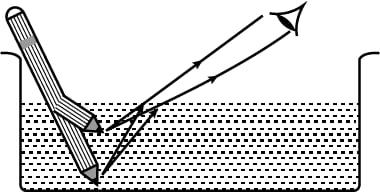#### Question 8

An object placed in one medium when seen from the other medium, appears to be vertically shifted. Name two factors on which the magnitude of the shift depends and state how does it depend on them.

The shift by which the object appears to be raised depends on —

(i) The refractive index of the medium — Higher the refractive index of the medium, more is the shift.

(ii) The thickness of the denser medium — Thicker the medium, more is the shift.

(iii) The colour or wavelength of incident light — the shift decreases with the increase in the wavelength of light used.

## Multiple Choice Type

#### Question 1

A small air bubble in a glass block when seen from above appears to be raised because of —

1. refraction of light ✓
2. reflection of light
3. reflection and refraction of light
4. none of the above

#### Question 2

An object in a denser medium when viewed from a rarer medium appears to be raised. The shift is maximum for—

1. red light
2. violet light ✓
3. yellow light
4. green light

## Numericals

#### Question 1

A water pond appears to be 2.7 m deep. If the refractive index of water is 4/3, find the actual depth of the pond.

We know that,

Real depth = Apparent depth x Refractive index of water

Given,

Apparent depth = 2.7 m

$\text {Refractive index of the water} = \dfrac{4}{3} \\[0.5em]$

So, substituting the values in the formula we get,

$\text {Real depth} = 2.7 \times \dfrac{4}{3} \\[0.5em] \Rightarrow \text {Real depth} = 3.6 m \\[0.5em]$

Hence, actual depth = 3.6 m

#### Question 2

A coin is placed at the bottom of a beaker containing water (refractive index = 4/3) at a depth of 12 cm. By what height the coin appears to be raised when seen from vertically above?

As we know,

$\text {Shift} = \text {Real depth} \times (1 – \dfrac{1}{_aμ_m})$

Given,

$\text {Refractive index of the water} = \dfrac{4}{3} \\[0.5em]$

and

Real depth = 12 cm

So, substituting the values in the formula we get,

$\text {Shift} = 12 \times (1 – \dfrac{3}{4}) \\[0.5em] \text {Shift} = 12 \times \dfrac{1}{4} \\[0.5em] \text {Shift} = \dfrac{12}{4} \\[0.5em] \Rightarrow \text {Shift} = 3 \\[0.5em]$

Hence, the coin appears to be raised by a height of 3 cm when seen from vertically above.

#### Question 3

A postage stamp kept below a rectangular glass slab of refractive index 1.5 when viewed from vertically above it, appears to be raised by 7.0 mm. Calculate the thickness of the glass slab.

As we know,

$\text {Shift} = \text {Real depth} \times (1 – \dfrac{1}{_aμ_m})$

Given,

Shift in the image = 7 mm or 0.7 cm

Refractive index of the glass block = 1.5

So, substituting the values in the formula we get,

$0.7 = \text {Real depth} \times (1 – \dfrac{1}{1.5}) \\[0.5em] 0.7 = \text {Real depth} \times \dfrac{0.5}{1.5} \\[0.5em] \text {Real depth} = \dfrac{0.7 \times 1.5}{0.5} \\[0.5em] \Rightarrow \text {Real depth} = 2.1 \\[0.5em]$

Hence, the thickness of glass slab = 2.1 cm.
(Thickness of glass slab is same as the real depth of the postage stamp).

## Exercise 4(D)

#### Question 1

Explain the term critical angle with the aid of a labelled diagram.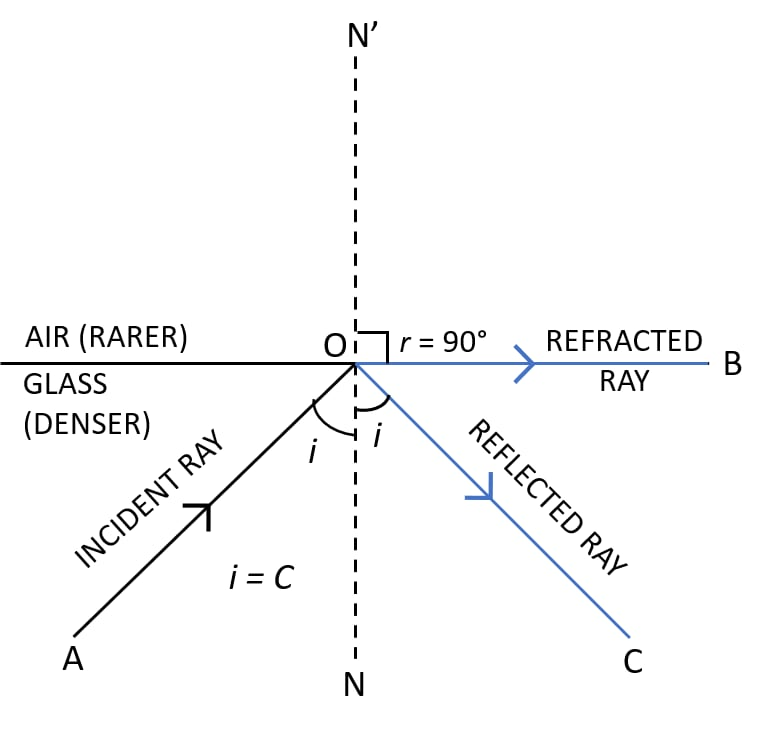When a ray of light passes from a denser to a rarer medium, at a certain angle of incidence i = C, the angle of refraction becomes 90°.

This angle C is called the critical angle.

Thus, critical angle is defined as the angle of incidence in the denser medium corresponding to which the angle of refraction in the rarer medium is 90°.

#### Question 2

How is the critical angle related to the refractive index of a medium?

Knowing the refractive index of the denser medium with respect to the rarer medium, we can calculate the critical angle C for that pair of medium with the help of the relation

$_a\mu_g = \dfrac{1}{\text {sin C}} \\[0.5em] = \text {cosec C}$

#### Question 3

State the approximate value of the critical angle for

(a) glass-air surface

(b) water-air surface.

(a) As we know,

$_aμ_g = \dfrac{1}{\text {sin C}} \\[0.5em] = \text {cosec C}$

Refractive index is

$_aμ_g = \dfrac{3}{2}$

Substituting the values in the formula we get,

$\dfrac{3}{2} = \dfrac{1}{\text {sin C}} \\[1em] \text {sin C} = \dfrac{1}{\dfrac{3}{2}} \\[1em] \text {sin C} = \dfrac{2}{3}$

As,

$\text {sin 42°} = \dfrac {2}{3}$

Hence, critical angle for glass air surface is 42°.

(b) As we know,

$_aμ_w = \dfrac{1}{\text {sin C}} = \text {cosec C} \\[0.5em]$

Refractive index is

$_aμ_w = \dfrac {4}{3} \\[0.5em]$

Substituting the values in the formula we get,

$\dfrac{4}{3} = \dfrac{1}{\text {sin C}} \\[1em] \text {sin C} = \dfrac{1}{\dfrac{4}{3}} \\[1em] \text {sin C} = \dfrac{3}{4} \\[1em] \text {sin 49°} = \dfrac{3}{4} \\[0.5em]$

Hence, critical angle for water air surface is 49°.

#### Question 4

What is the meant by the statement ‘the critical angle for diamond is 24°'?

The statement 'the critical angle for diamond is 24°' implies that at an incident angle of 24°, the angle of refraction in the air will be 90° within the diamond.

If the incident angle is more than the critical angle then the ray will suffer total internal reflection without any refraction.

#### Question 5

A light ray is incident from a denser medium on the boundary separating it from a rarer medium at an angle of incidence equal to the critical angle. What is the angle of refraction for the ray?

The angle of refraction becomes equal to 90° when a light ray is incident from a denser medium on the boundary separating it from a rarer medium at an angle of incidence equal to the critical angle. Below diagram shows the path of the ray when angle of incidence is equal to the critical angle:#### Question 6

Name two factors which affect the critical angle for a given pair of media. State how do the factors affect it.

The critical angle depends on two factors:

(a) The colour or wavelength of light — The refractive index of a transparent medium decreases with the increase of wavelength of light. (It is most for violet light and least for red light), therefore the critical angle for a pair of media is least for the violet light and most for the red light, i.e. the critical angle increases with the increase in wavelength of light.

(b) The temperature — On increasing the temperature of medium, its refractive index decreases. So, the critical angle for the pair of medium increases with increase in temperature.

#### Question 7

The critical angle for glass-air is 45° for the light of yellow colour. State whether it will be less than, equal to, or more than 45° for

(i) red light,

(ii) blue light ?

As the wavelength of light decreases (or increases) refractive index becomes more (or less) and critical angle becomes less (or more).

(i) As the wavelength for red light is more than that for yellow colour, the refractive index becomes less and the critical angle will be more than 45°.

(ii) As the wavelength for blue light is less than that for yellow colour, the refractive index becomes more and the critical angle will be less than 45°.

#### Question 8

Which colour of light has higher critical angle ? Red light or green light.

As we know, the relation between critical angle and refractive index is

$\text {sin c} = \dfrac{1}{μ}$

where, c is critical angle and μ is refractive index.

As μ for red light is less as compared to that for green light and μ and c are inversely related.

Hence, the critical angle of red light is higher than that of green light.

#### Question 9

(a) What is total internal reflection?

(b) State two conditions necessary for total internal reflection to occur.

(c) Draw diagrams to illustrate critical angle and total internal reflection.

(a) When a ray of light travelling in a denser medium, is incident at the surface of a rarer medium at the angle of incidence greater than the critical angle for the pair of media, the ray is totally reflected back into the denser medium. This phenomenon is known as total internal reflection.

(b) The two necessary conditions for total internal reflection are —

1. The light must travel from a denser medium to a rarer medium.
2. The angle of incidence must be greater than the critical angle for the pair of media.

(c) Below diagrams illustrate critical angle and total internal reflection:

Critical Angle Diagram:Total Internal Reflection Diagram: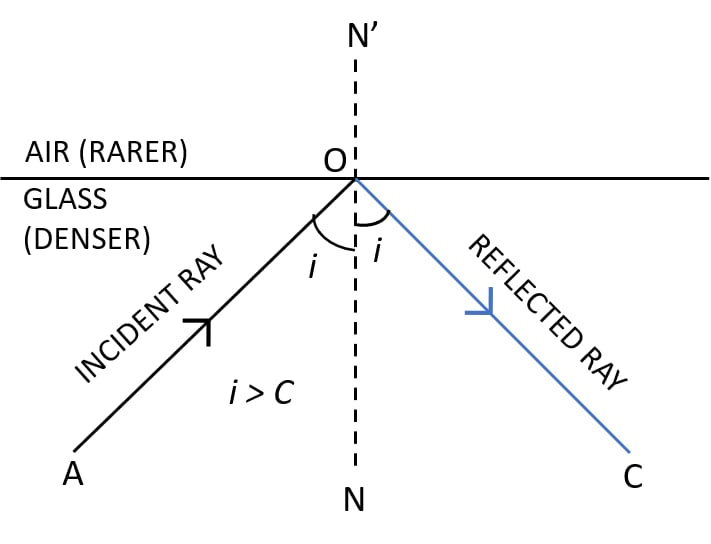#### Question 10

Fill in the blanks to complete the following sentences —

(a) Total internal reflection occurs only when a ray of light passes from a .......... medium to a .......... medium.

(b) Critical angle is the angle of .......... in denser medium for which the angle of .......... in rarer medium is ..........

(a) Total internal reflection occurs only when a ray of light passes from a denser medium to a rarer medium.

(b) Critical angle is the angle of incidence in denser medium for which the angle of refraction in rarer medium is 90°.

#### Question 11

State whether the following statement is true or false —

If the angle of incidence is greater than the critical angle, light is not refracted at all, when it falls on the surface from a denser medium to a rarer medium.

True

#### Question 12

The refractive index of air with respect to glass is expressed as

$_gμ_a = \dfrac {\text {sin i}}{\text {sin r}}$

(a) Write down a similar expression for aμg in terms of the angles i and r.

(b) If angle r = 90°, what is the corresponding angle i called?

(c) What is the physical significance of the angle i in part (b)?

(a) The expression for aμg = $\dfrac {\text {sin r}}{\text {sin i}}$

(b) When refractive angle r = 90° then, the corresponding angle of incidence i will be equal to critical angle.

(c) The phenomenon of total internal reflection occurs when the angle of incidence exceeds the value of i obtained in part (b).

#### Question 13

Figure below shows two rays A and B travelling from water to air. If the critical angle for water-air surface is 48°, complete the ray diagram showing the refracted rays for each. State conditions when the ray will suffer total internal reflection.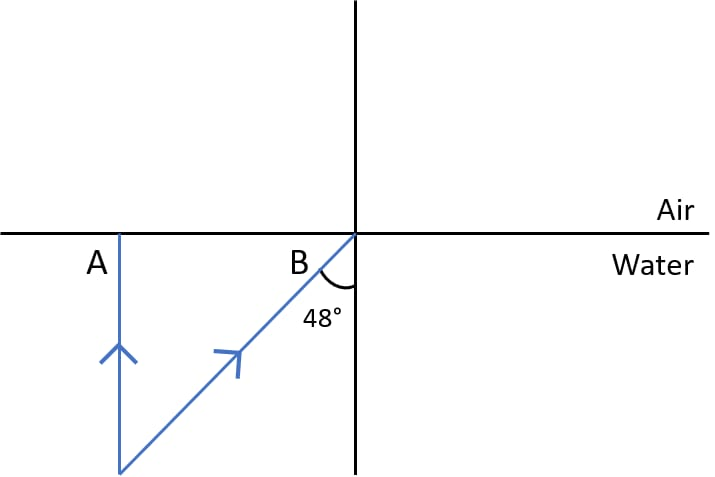Completed ray diagram with refracted rays labelled is shown below: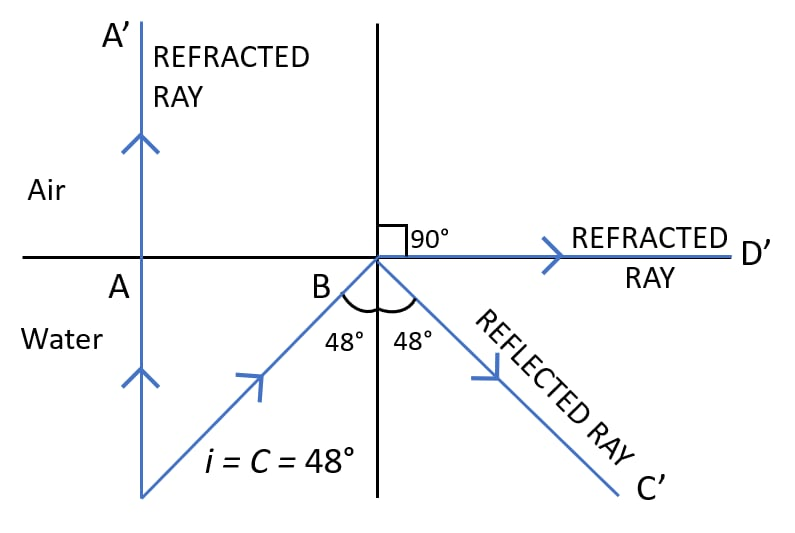Ray A is incident normally at water air boundary so its angle of incidence is 0°. Hence, the refracted ray shown as AA' in the diagram passes undeviated.

For Ray B, the angle of incidence is equal to the critical angle for water air boundary so it is partly reflected as BC' and partly refracted as BD'.

Two necessary conditions for the total internal reflection are —

1. The light must travel from a denser to a rarer medium.
2. The angle of incidence must be greater than the critical angle for the pair of medium.

#### Question 14

Figure shows a point source P inside a water container. Three rays A, B and C starting from the source P are shown up to the water surface.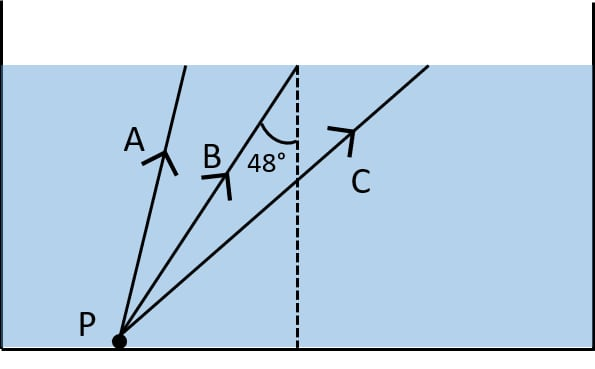(a) Show in the diagram, the path of these rays after striking the water surface. The critical angle for water-air surface is 48°.

(b) Name the phenomenon which the rays A, B and C exhibit.

(a) Below diagram shows the path of the rays after striking the water surface: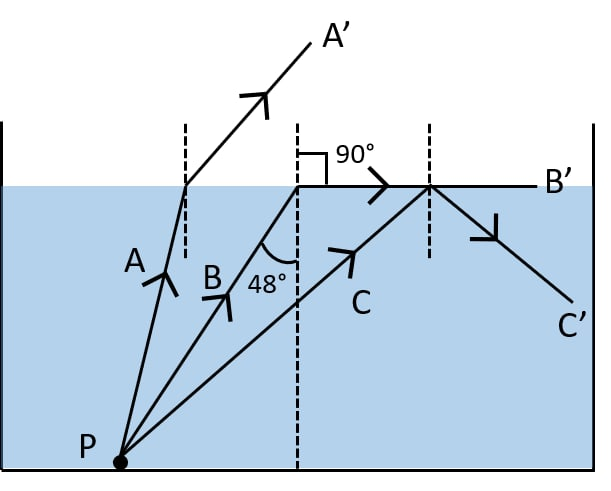(b) Rays A and B show the phenomenon of ‘refraction of light’ and ray C shows the phenomenon of ‘total internal reflection’.

#### Question 15

In the figure, PQ and PR are the two light rays emerging from an object P. The ray PQ is refracted as QS.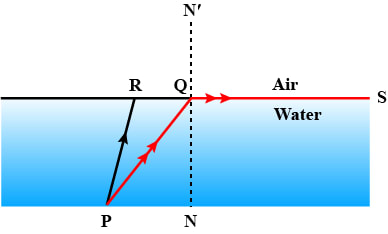(a) State the special name given to the angle of incidence ∠PQN of the ray PQ.

(b) What is the angle of refraction for the refracted ray QS?

(c) Name the phenomenon that occurs if the angle of incidence ∠PQN is increased.

(d) The ray PR suffers partial reflection and refraction on the water-air surface. Give reason.

(e) Draw in the diagram the refracted ray for the incident ray PR and hence show the position of image of the object P by the letter P' when seen vertically from above.

(a) The angle of incidence ∠PQN is known as the critical angle.

(b) For the refracted ray QS, the angle of refraction is 90°.

(c) When the angle of incidence ∠PQN is increased, then the phenomenon that occurs is total internal reflection.

(d) For the ray PR, the angle of incidence is less than ∠PQN ( i.e the critical angle ). So according to the laws of reflection, ray PR suffers partial reflection and refraction.

(e) Below diagram shows the refracted ray and the position of image of the object P when seen vertically from above: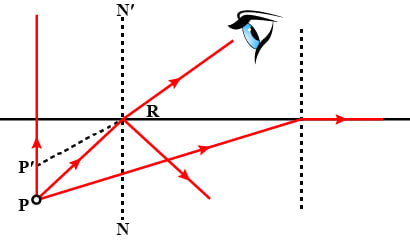#### Question 16

The refractive index of glass is 1.5. From a point P inside a glass slab, draw rays PA, PB and PC incident on the glass-air surface at an angle of incidence 30°, 42° and 60° respectively.

(a) In the diagram show the approximate direction of these rays as they emerge out of the slab.

(b) What is the angle of refraction for the ray PB?

(Take sin 42° = 2 / 3)

(a) Diagram with rays PA, PB and PC is shown below: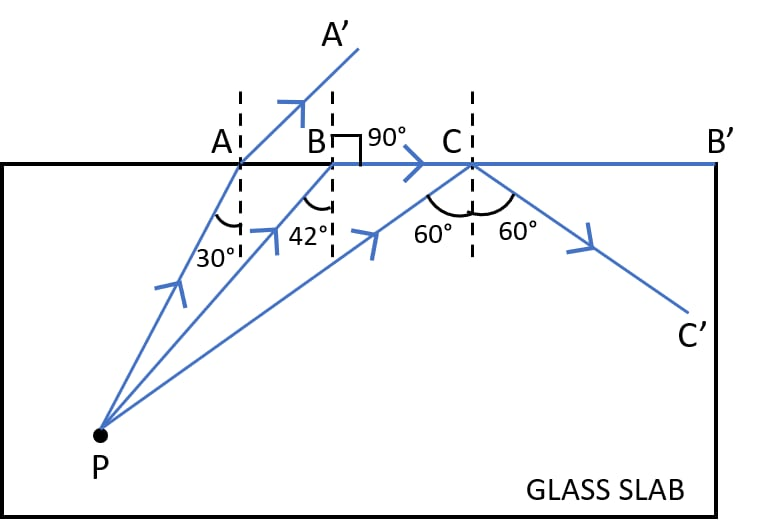(b) Given,

μg = 1.5

As we know,

$\text {sin i}_c = \dfrac {1}{μ} \\[0.5em]$

Substituting the values in the formula we get,

$\text {sin i}_c = \dfrac{1}{1.5} \\[0.5em] \Rightarrow \text {sin i}_c = 0.667 \\[0.5em] \Rightarrow i_c = 41.8$

Hence, we can round off ic = 42°

Applying,

$\dfrac{\text {sin r}}{\text {sin i}} = _a\mu_g \\[0.5em] \text {sin r} = _a\mu_g \times \text {sin i} \\[0.5em] \text {sin r} = _a\mu_g × \text {sin 42°} \\[0.5em]$

Given,

$\text {sin 42°} = \dfrac{2}{3}$

and

$_aμ_g = \dfrac{3}{2}$

Substituting the values in the formula we get,

$\text{sin r} = \dfrac{3}{2} \times \dfrac{2}{3} \\[0.5em] \Rightarrow \text {sin r} = 1 \\[0.5em]$

As sin r = 1 , so angle of refraction = 90°.

#### Question 17

A ray of light enters a glass slab ABDC as shown in figure and strikes at the centre O of the circular part AC of the slab. The critical angle of glass is 42°. Complete the path of the ray till it emerges out from the slab. Mark the angles in the diagram wherever necessary.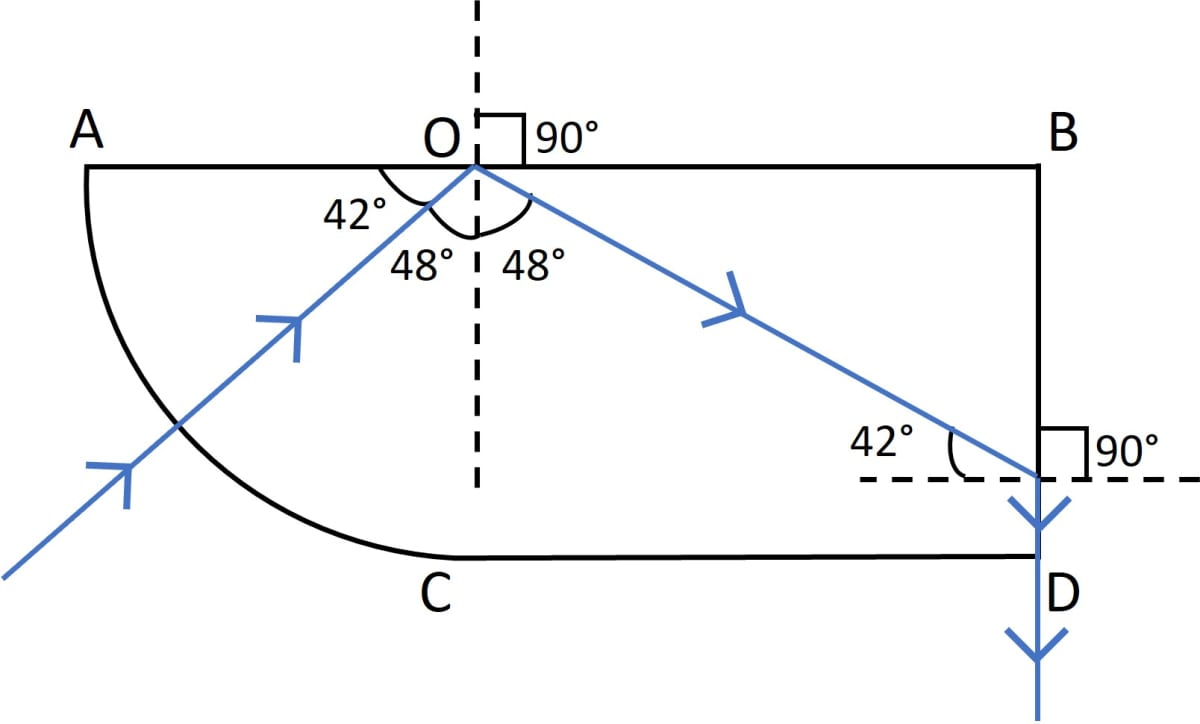Completed diagram showing the path of the ray till it emerges out from the slab is shown below: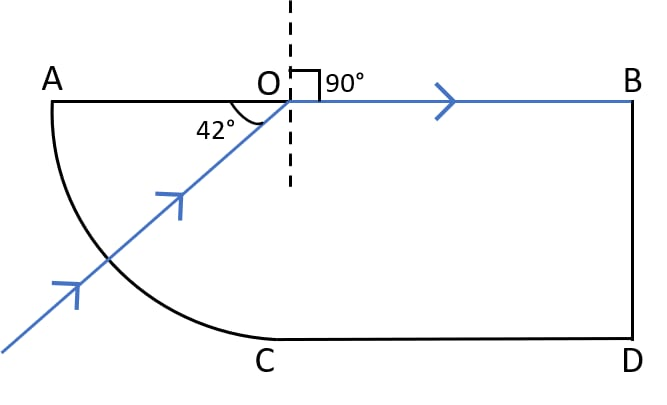#### Question 18

What is a total reflecting prism? State three actions that it can produce. Draw a diagram to show one such action of the total reflecting prism.

A prism having an angle of 90° between its two refracting surfaces and the other two angles each equal to 45° is called a total reflecting prism. In such a prism, the light incident normally on any of its faces suffers total internal reflection inside the prism.

Due to this property, a total reflecting prism is used for the following three purposes —

1. To deviate a ray of light through 90°.
2. To deviate a ray of light through 180°.
3. To erect the inverted image without producing deviation in its path.

Below diagram shows the deviation of a ray of light through 90° by a total reflecting prism: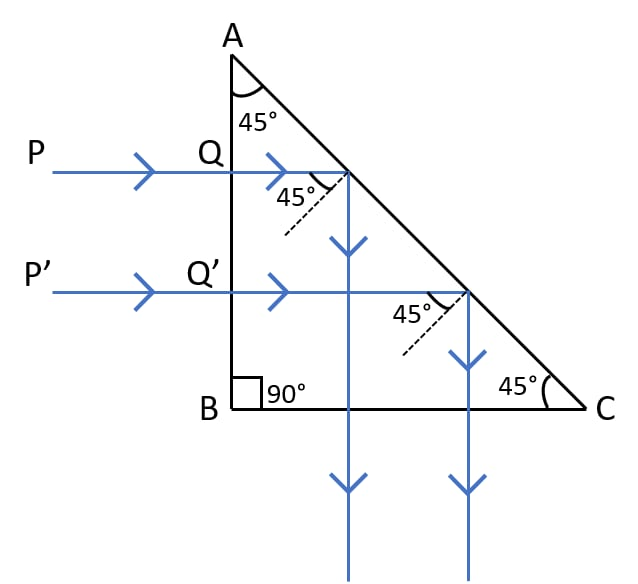#### Question 19

Show with the help of a diagram how a total reflecting prism can be used to turn a ray of light through 90°. Name one instrument in which such a prism is used.

Below diagram shows the deviation of a ray of light through 90° by a total reflecting prism:ABC is a total reflecting prism. A beam of light is incident normally at the face AB. It passes undeviated into the prism and strikes at the face AC making an angle of incidence equal to 45°.

For glass air interface, the critical angle is equal to 42°, therefore the beam of light suffers total internal reflection at the face AC because the angle of incidence is greater than the critical angle.

The reflected beam inside the prism then strikes the face BC, where it is incident normally and therefore passes undeviated. Hence, the incident beam gets deviated through 90°.

This action of prism is used in a periscope where a total reflecting prism is preferred over a plane mirror.

#### Question 20

A ray of light XY passes through a right-angled isosceles prism as shown in the adjacent diagram.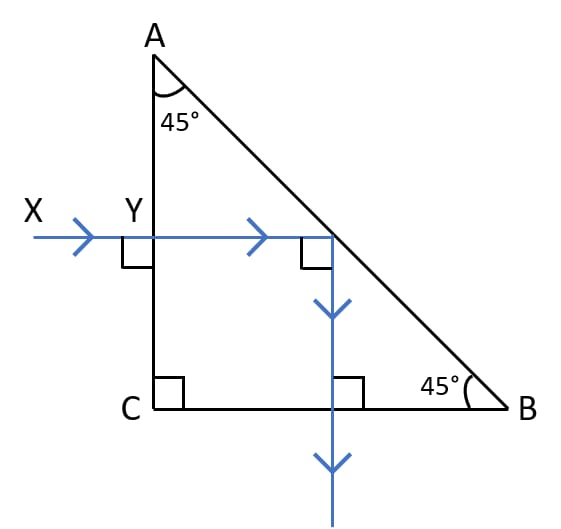(a) What is the angle through which the incident ray deviates and emerges out of the prism?

(b) Name the instrument where this action of prism is put into use.

(c) Which prism surface will behave as a mirror?

(a) The angle through which the incident ray deviates and emerges out of the prism is the angle of deviation.

The angle of deviation = the angle which the emergent ray makes with the incident ray XY = 90°.

(b) The instrument where this action of prism is put into use is the periscope.

(c) The surface AB of the prism behaves as a mirror.

#### Question 21

Draw a diagram of a right angled isosceles prism which is used to make an inverted image erect.

Below diagram shows the usage of a right angled isosceles prism which is used to make an inverted image erect: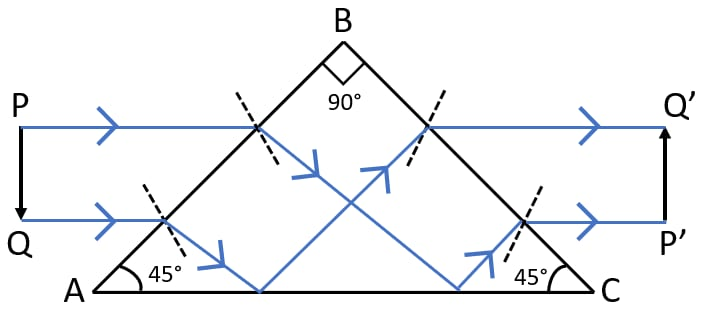This action of prism is used in a slide.

#### Question 22

In the figure, a ray of light PQ is incident normally on the hypotenuse of an isosceles right angled prism ABC.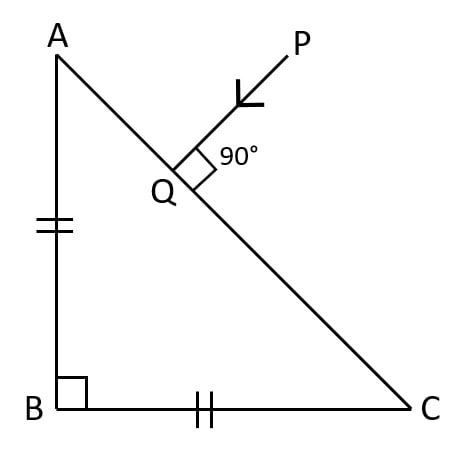(a) Complete the path of the ray PQ till it emerges from the prism. Mark in the diagram the angle wherever necessary.

(b) What is the angle of deviation of the ray PQ?

(c) Name a device in which this action is used.

(a) Below diagram shows the path of the ray PQ till it emerges from the prism with all the angles labelled: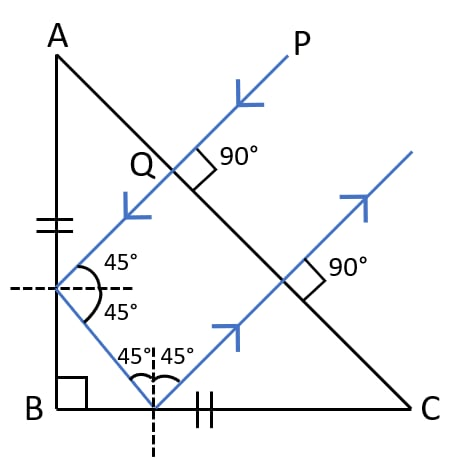(b) The angle of deviation of the ray PQ is 180°.

(c) This action of the prism is used in Binoculars.

#### Question 23

In the figure given below, a ray of light PQ is incident normally on the face AB of an equilateral glass prism. Complete the ray diagram showing its emergence into air after passing through the prism. Take critical angle for glass = 42°.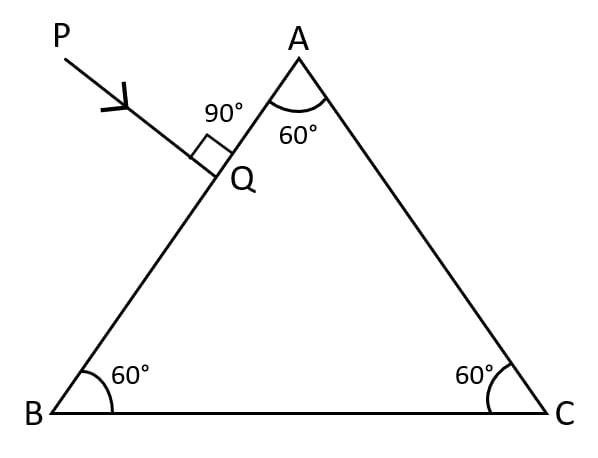(a) Write the angles of incidence at the faces AB and AC of the prism.

(b) Name the phenomenon which the ray of light suffers at the face AB, AC and BC of the prism.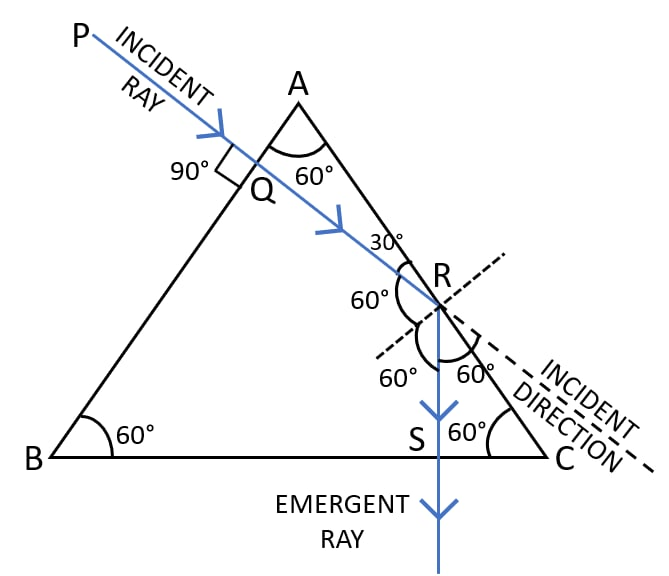(a) The angle of incidence at the faces AB, i = 0°.
The angle of incidence at the faces AC, i = 60°.

(b) The phenomenon which the ray of light suffers at:
face AB — refraction
face AC — total internal reflection
face BC — refraction

#### Question 24

Copy the diagram given below and complete the path of the light ray till it emerges out of the prism. The critical angle of glass is 42°. In your diagram mark the angles wherever necessary.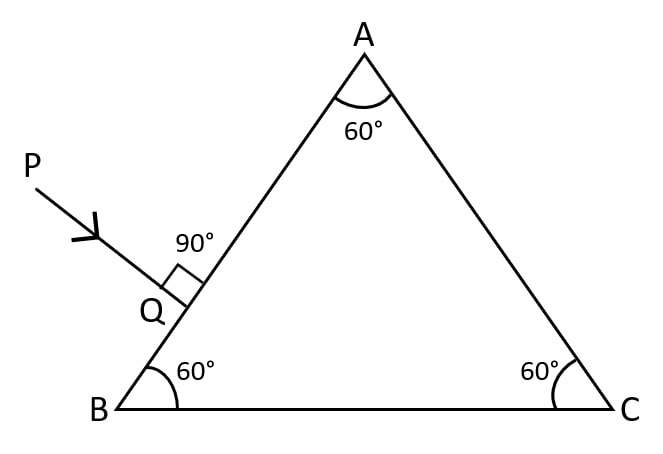Below is the completed diagram showing the path of the light ray till it emerges out of the prism with all angles marked: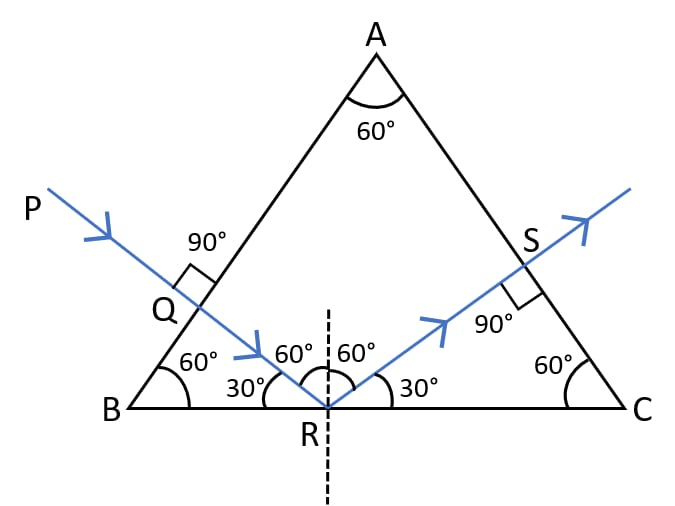#### Question 25

Draw a neat labelled ray diagram to show total internal reflection of a ray of light incident normally on one face of a 30°, 90°, 60° prism.

Below is the labelled ray diagram showing total internal reflection of a ray of light incident normally on one face of a 30°, 90°, 60° prism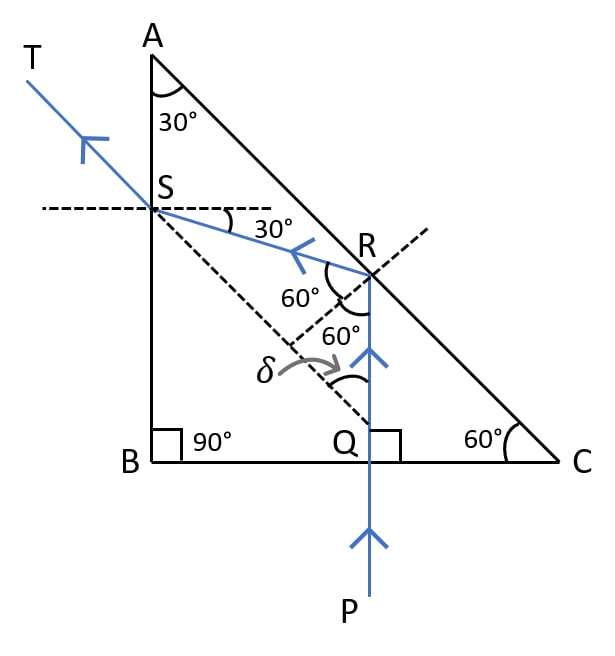#### Question 26

What device other than a plane mirror, can be used to turn a ray of light through 180°? Draw a diagram in support of your answer. Name an instrument in which this device is used.

A total reflecting prism is used to turn a ray of light through 180°.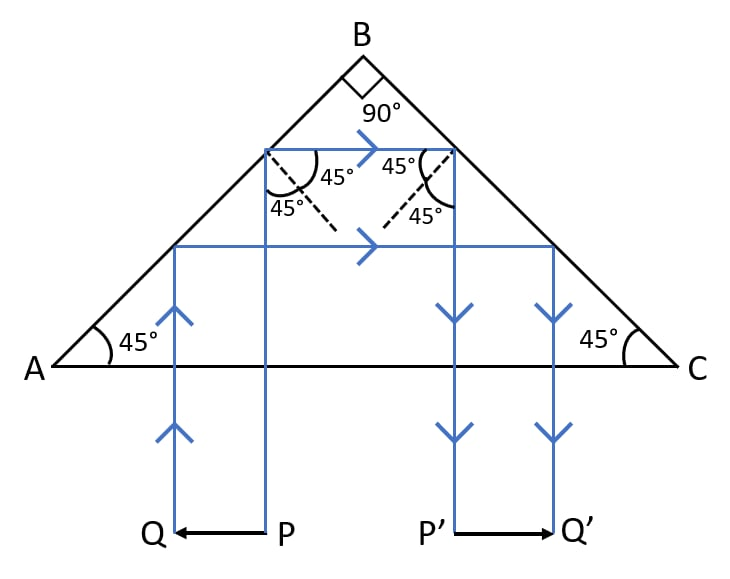This device is used in a binocular and camera to invert the image without the loss of intensity.

#### Question 27

Mention one difference between the reflection of light from a plane mirror and total internal reflection of light from a prism.

Plane mirrorPrism
In reflection of light from a plane mirror, only a part of the light is reflected while rest is refracted and absorbed. So, the reflection is partial.In total internal reflection of light from a prism, the entire incident light is reflected back into the denser medium. So there is no loss of light energy.

#### Question 28

State one advantage of using a total reflecting prism as a reflector in place of a plane mirror.

The advantage of using a total reflecting prism as a reflector in place of a plane mirror, is that the image is much brighter and the brightness remains unchanged even after the long use of the total reflecting device.

In the case of a plane mirror, the image is less bright and the brightness gradually decreases as the silvering on mirror becomes old and rough.

#### Question 29

Two isosceles right-angled glass prisms P and Q are placed near each other as shown in figure. Complete the path of the light ray entering the prism P till it emerges out of the prism Q.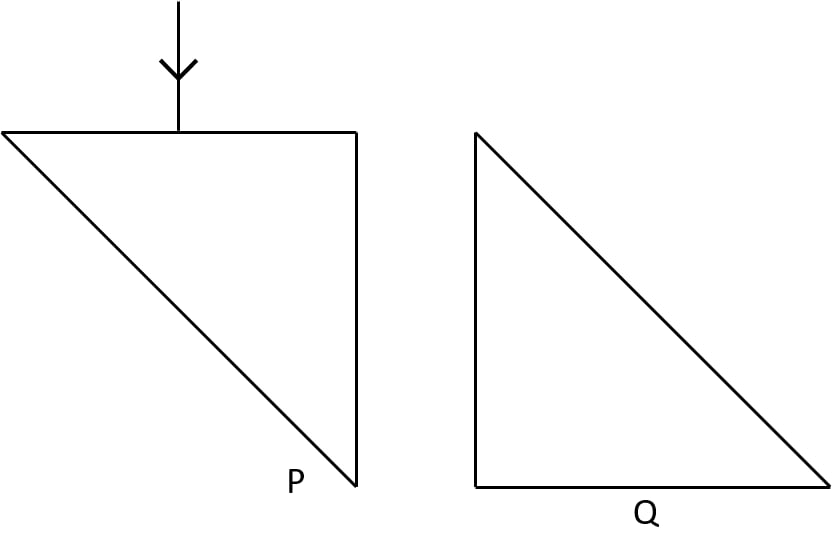Below diagram shows the path of the light ray entering the prism P till it emerges out of the prism Q: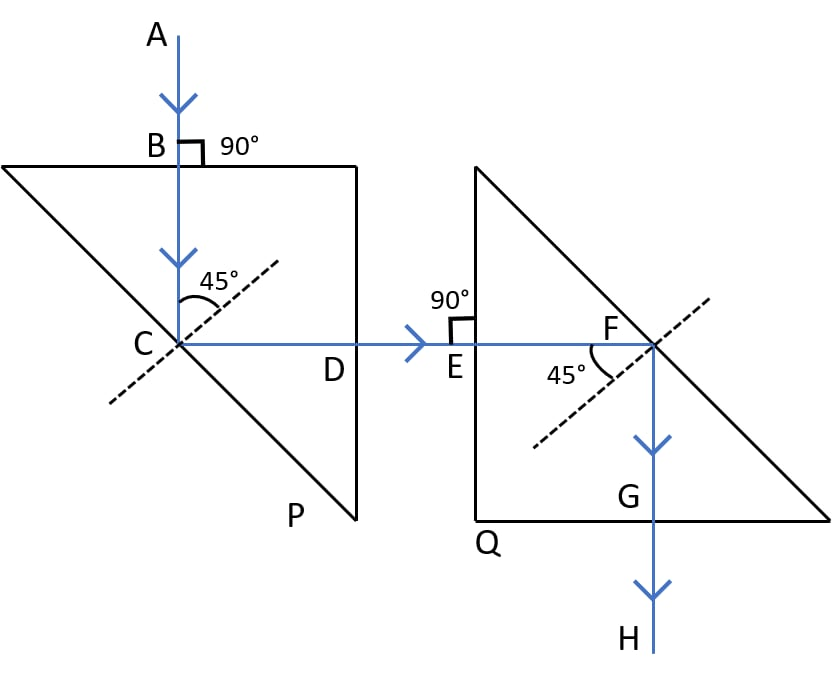#### Question 30

Complete the path of ray PQ through the glass prism ABC as shown in figure till it emerges out of the prism. Given the critical angle of glass is 42°.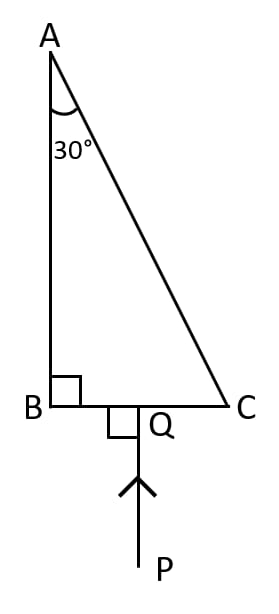Below diagram shows the path of ray PQ through the glass prism ABC till it emerges out of the prism: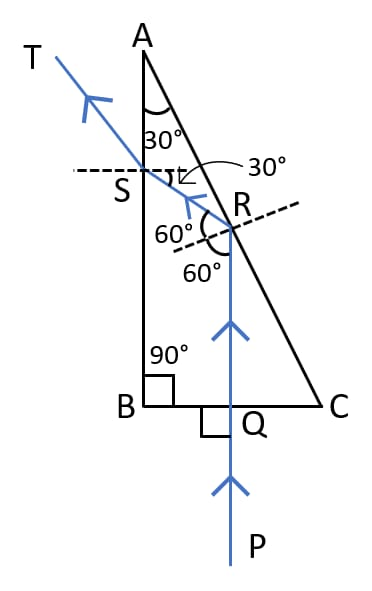## Multiple Choice Type

#### Question 1

The critical angle for the glass-air interface is:

1. 24°
2. 48°
3. 42° ✓
4. 45°

#### Question 2

A total reflecting right-angled isosceles prism can be used to deviate a ray of light through:

1. 30°
2. 60°
3. 75°
4. 90° ✓

#### Question 3

A total reflecting equilateral prism can be used to deviate a ray of light through:

1. 30°
2. 60° ✓
3. 75°
4. 90°